Home

ARTICLE 43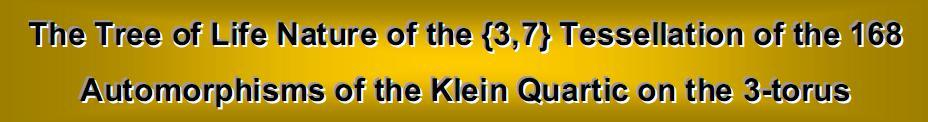by

Stephen M. Phillips

Flat 4, Oakwood House, 117-119 West Hill Road. Bournemouth. Dorset BH2 5PH. England.

Website: http://www.smphillips.mysite.com

Abstract

 The Godnames assigned to the ten Sephiroth of the Tree of Life prescribe properties of the dodecagon. As the tenth regular polygon, the dodecagon is unique in embodying the superstring structural parameter 168, the dimension 248 of the superstring gauge symmetry group E8 and the number of chromosomes in the human cell. Moreover, its geometrical composition conforms to the 3:7 pattern of yods in the tetractys — the Pythagorean counterpart of the Tree of Life. The 24 hexagonal yods on its edges and the 144 internal yods surrounding its centre denote, respectively, the 24 leptons and 144 subquarks belonging to the family of 168 particles hypothesized in Part 4 of Article 40. Six 7-fold systems exhibiting different kinds of duality and characterized by the number 168 are compared. The basic 24:144 division of any holistic system is displayed in the 168 yods other than corners associated with each set of the first six enfolded polygons of the inner Tree of Life, the pentagon having 24 such yods and the five other polygons having 144 yods. The same division appears in the two separate Type B dodecagons, which have 24 corners and 144 vertices and edges of their 72 tetractyses. It also manifests in the disdyakis triacontahedron, whose properties are analogous to the {3,7} tessellation of the 3-torus. Constructed from four triangular prisms and six square antiprisms, the 3-torus is composed of 260 geometrical elements. This indicates that it conforms to the archetypal pattern of the inner form of the Tree of Life, whose seven enfolded polygons contain 260 yods outside their shared edge. The Sri Yantra has the same geometrical composition. Just as 336 yods are on the edges of the separate prisms and antiprisms, so 336 yods line the edges of the 42 triangles of the Sri Yantra when they are turned into tetractyses. Their counterparts in the pair of joined dodecagons are the 336 yods other than their corners. In each case, they denote the number of circularly polarized oscillations of each closed curve of the heterotic superstring as it winds once around triangular faces are each divided into three triangles. Additional evidence that the tessellated 3-torus conforms to the Tree of Life is the fact that 264 hexagonal yods lie on the edges of the 56 triangular faces of the separate prisms and antiprisms, for this is the number of yods in the seven enfolded polygons. Alternatively, the separate prisms and antiprisms of the 3-torus and its turned inside-out version have 264 edges when their triangular faces are divided into three tetractyses. The 3-torus with single tetractyses as faces comprises 248 yods that denote the 248 roots of E8, the yods at the centres of the eight triangular faces of the four prisms denoting its eight simple roots. The two halves of the Sri Yantra are the counterpart of the 3-torus and its turned inside-out version. Each half of the first two layers has nine triangles corresponding to the four prisms. Each half of the last two layers with 12 triangles corresponds to the six antiprisms. 192 yods line the edges of the 56 triangles of the {3,7} tessellation when they are turned into tetractyses. 24 of them are vertices of the prisms and the other 168 are hexagonal yods on the 48 edges of the antiprisms. Their counterpart in the Pythagorean musical scale are the 168 harmonics between the 10th overtone with tone ratio 24 and the 24th overtone with tone ratio 192. 1680 yods surround the vertices and centres of the 56 triangles in the tessellated 3-torus when their 168 sectors are each divided into three tetractyses. The {3,7} tessellation embodies not only the dimension 248 of the superstring symmetry group E8 but also the superstring structural parameter 1680 — the number of circularly polarized oscillations in each closed curve of the E8×E8 heterotic superstring

1

Table 1. Gematria number values of the ten Sephiroth in the four Worlds.

 SEPHIRAH GODNAME ARCHANGEL ORDER OF ANGELS MUNDANE CHAKRA 1 Kether (Crown)                  620 EHYEH (I am)                  21 Metatron (Angel of the Presence)                  314 Chaioth ha Qadesh (Holy Living Creatures)                  833 Rashith ha Gilgalim First Swirlings. (Primum Mobile)                  636 2 Chokmah (Wisdom)                  73 YAHWEH, YAH (The Lord)                  26, 15 Raziel (Herald of the Deity)                  248 Auphanim (Wheels)                  187 Masloth (The Sphere of the Zodiac)                  140 3 Binah (Understanding)                  67 ELOHIM (God in multiplicity)                  50 Tzaphkiel (Contemplation of God)                  311 Aralim (Thrones)                  282 Shabathai Rest. (Saturn)                  317 Daath (Knowledge)                  474 4 Chesed (Mercy)                  72 EL (God)                  31 Tzadkiel (Benevolence of God)                  62 Chasmalim (Shining Ones)                  428 Tzadekh Righteousness. (Jupiter)                  194 5 Geburah (Severity)                  216 ELOHA (The Almighty)                  36 Samael (Severity of God)                  131 Seraphim (Fiery Serpents)                  630 Madim Vehement Strength. (Mars)                  95 6 Tiphareth (Beauty)                  1081 YAHWEH ELOHIM (God the Creator)                  76 Michael (Like unto God)                  101 Malachim (Kings)                  140 Shemesh The Solar Light. (Sun)                  640 7 Netzach (Victory)                  148 YAHWEH SABAOTH (Lord of Hosts)                  129 Haniel (Grace of God)                  97 Tarshishim or Elohim                  1260 Nogah Glittering Splendour. (Venus)                  64 8 Hod (Glory)                  15 ELOHIM SABAOTH (God of Hosts)                  153 Raphael (Divine Physician)                  311 Beni Elohim (Sons of God)                  112 Kokab The Stellar Light. (Mercury)                  48 9 Yesod (Foundation)                  80 SHADDAI EL CHAI (Almighty Living God)                  49, 363 Gabriel (Strong Man of God)                  246 Cherubim (The Strong)                  272 Levanah The Lunar Flame. (Moon)                  87 10 Malkuth (Kingdom)                  496 ADONAI MELEKH (The Lord and King)                  65, 155 Sandalphon (Manifest Messiah)                  280 Ashim (Souls of Fire)                  351 Cholem Yesodoth The Breaker of the Foundations. The Elements. (Earth)                  168

 The Sephiroth exist in the four Worlds of Atziluth, Beriah, Yetzirah and Assiyah. Corresponding to them are the Godnames, Archangels, Order of Angels and Mundane Chakras (their physical manifestation). This table lists their number values obtained by the practice of gematria, wherein a number is assigned to each letter of the alphabet, thereby giving to a word a number value that is the sum of the numbers associated with its letters.

(All numbers from this table referred to in the article are written in boldface).

2

1. Godnames prescribe the dodecagon

The Godnames (Table 1) prescribe the dodecagon and pair of dodecagons as follows:
EHYEH (21):
number of yods in Type A dodecagon = 73 = 21st prime number.
YAH (15): number of yods in the root edge and pair of Type A dodecagons = 73 + 4 + 73 = 150 = 10×15.
YAHWEH (26): number of vertices of 24 sectors in pair of separate Type A dodecagons = 26. Number of geometrical elements in pair of Type A dodecagons + root edge = 98 + 3 = 101 = 26th prime number. 260 (=26×10) yods in two joined dodecagons outside root edge other than vertices & centres of tetractyses.
ELOHIM (50): number of vertices & triangles in pair of separate Type A dodecagons = 26 + 24 = 50. Number of corners of 72 tetractys sectors of pair of Type B dodecagons = 50. There are 282 yods on the 118 external edges of the two separate Type B dodecagons. 282 is the number value of Aralim (“Thrones”), the Order of Angels assigned to Binah.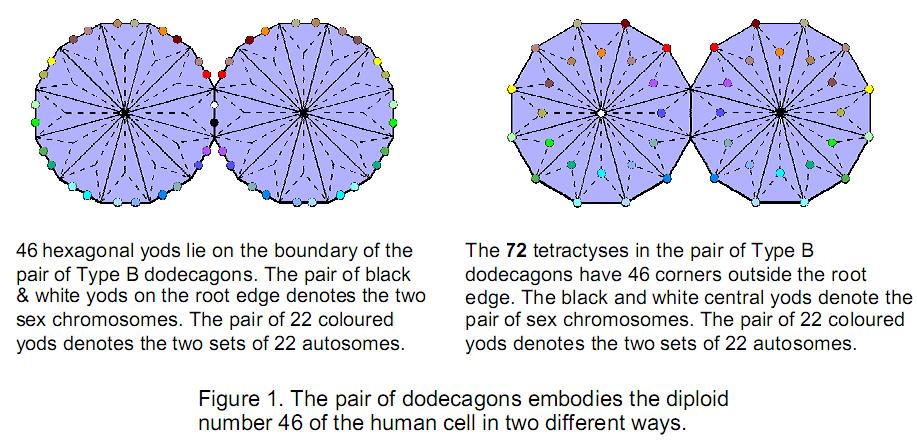EL (31): number of yods on edges of 12 tetractyses in Type A dodecagon = 61 = 31st odd integer. 310 (=31×10) hexagonal yods in pair of joined, Type B dodecagons.
ELOHA (36): number of yods on boundary of dodecagon = 36. Number of yods surrounding centres of pair of separate, Type B dodecagons = 360 = 36×10.
YAHWEH ELOHIM (76): number of yods on boundaries of two separate dodecagons and in root edge = 36 + 36 + 4 = 76. YAHWEH (26) prescribes their 26 corners and endpoints; ELOHIM (50) prescribes the number of hexagonal yods.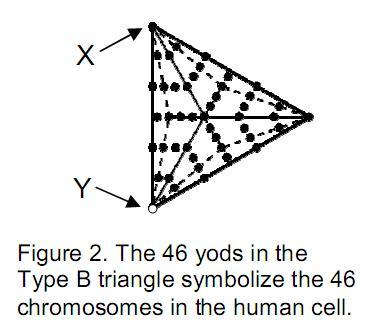YAHWEH SABAOTH (129): 130 yods outside the root edge in dodecagon other than vertices & centres of tetractyses. 130 = 129th integer after 1.
ELOHIM SABAOTH (153): 153 yods outside the root edge other than 24 yods on the internal edges of sectors.
EL ChAI (49): number of edges in root edge and pair of separate Type A dodecagons = 24 + 1 + 24 = 49. Number of vertices, edges & triangles in pair of separate Type A dodecagons = 26 + 48 + 24 = 98 = 49th even integer.
Number of vertices, edges & triangles in Type A dodecagon = 13 + 24 + 12 = 49.
ADONAI MELEKH (155): 155 hexagonal yods associated with each joined, Type B dodecagon.

3

Of the 181 yods in a dodecagon, 36 are centres of tetractyses, leaving 145 yods on their 60 edges. 141 yods are on edges outside the root edge. The pair of joined dodecagons has (141+4+141=286) yods on 61 edges. Of these, 46 yods are vertices of 72 tetractyses, leaving 240 hexagonal yods on edges. The pair of joined dodecagons has 358 yods. 46 of these are vertices of 72 tetractyses, leaving 312 hexagonal yods. The pair of joined dodecagons (Fig. 1) embodies the human diploid number 46. The X and Y sex chromosomes (non-autosomes) correspond to the centres of the two dodecagons. The 44 vertices outside the root edge correspond to the 44 autosomal chromosomes. Alternatively, there are 46 hexagonal yods on the boundaries of the two dodecagons, the pair of hexagonal yods on the root edge symbolizing the X and Y chromosomes. The 46 yods in a Type B triangle (Fig. 2) also symbolize the 46 human chromosomes, the sex chromosomes corresponding to the two ends of the root edge.

2. 240 roots of E8

A Type B dodecagon has 25 vertices, 60 edges & 36 triangles. 84 vertices & edges surround its centre. The pair of separate dodecagons has (84+84=168) vertices & edges surrounding their centres. Each dodecagon has 181 yods, i.e., 168 yods other than its centre and corners. The two separate dodecagons have 72 triangles. There are 240 geometrical elements surrounding the centres of the pair of dodecagons. The split:

240 = 168 + 72

mirrors the division between the 72 roots of E6, a subgroup of the superstring gauge symmetry group E8, and the 168 roots of E8 that are not roots of E6. It corresponds to the 168 vertices & edges and the 72 triangles surrounding the centre of the dodecagon.

This 72:168 division appears, alternatively, as follows: a Type A dodecagon has 13 vertices and 24 edges of its 12 sectors, i.e. 49 geometrical elements. The 240 geometrical elements of the two Type B dodecagons comprise 72 elements found in the pair of Type A dodecagons and 168 extra elements. Their composition is:

(12+12)×(1 vertex+3 edges+3 triangles) = 24 vertices+(3×24=72) edges+(3×24=72) triangles

The numbers of the three types of geometrical element are displayed below:

Number of vertices (V), edges (E) & triangles (T) surrounding centre of dodecagon

 V E F Total Type A: 12 (24) 24 (48) 12 (24) 48 (96) Type B: 24 (48) 60 (120) 36 (72) 120 (240)

(Numbers in brackets refer to the pair of dodecagons). Surrounding the centres of the pair of Type A dodecagons are 24 vertices & triangles and 24 edges. This 24:24 division of the 48 elements is universally found in holistic systems.

The 72:168 division appears in the tetractys representation of 240: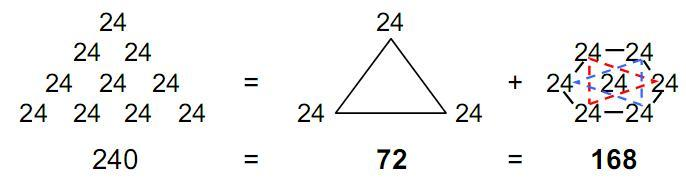The triplet of 24s at the corners of the triangle corresponds to the 24 corners of the two Type A dodecagons, the 24 boundary edges and the 24 radial edges of their sectors. The central 24 corresponds to the 24 centres of the sectors of the dodecagons. One triplet of 24s (blue dashed triangle) correspond to the (3×24) edges inside the sectors

4

and the other triplet of 24s (red dashed triangle) corresponds to the (3×24) triangles.

A separate Type A and a Type B dodecagon have 168 geometrical elements surrounding their centres. They comprise 84 edges and 84 vertices & triangles. This 84:84 division is intrinsic to holistic systems. The joined pair of dodecagons has 248 yods surrounding their centres. They symbolize the 248 roots of E8.

3. Comparison with 168 particles

It was hypothesized in Article 40 (Part 4) that the family of supersymmetric particles consists of 168 members. They comprise 12 leptons & 12 anti-leptons, 72 subquarks & 72 anti-subquarks. They correspond in the Type B dodecagon (Fig. 3) to the 12 pairs of yods on its boundary, the 72 hexagonal yods on 36 edges of the 36 tetractyses forming Y shapes and the 72 yods that are either hexagonal yods on radial edges, centres of tetractyses or centres of sectors: 168 = 12×(2+12). The 14 yods per sector comprise the two hexagonal yods on the boundary of the dodecagon and the 12 internal yods.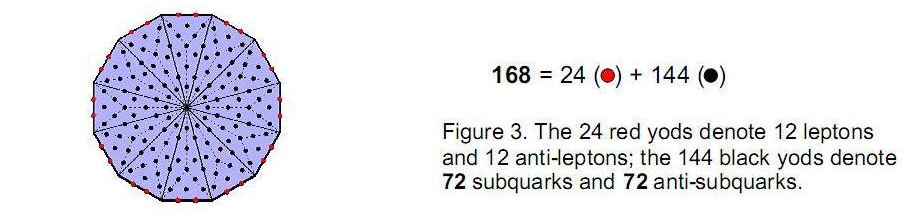The counterpart of this in acupuncture is the distinction between the 12 acupoint meridians and the Governing and Conception Vessels. It also mirrors the distinction between the two simple roots (α0, α0) of G2, the group of automorphisms of the Fano plane, and its 12 roots forming four triplets arranged three-dimensionally as two nested Stars of David. Finally, it corresponds to the 14 types of notes making up the seven musical scales: the tonic, octave, and the 12 notes between them. As 168 = 12×(2+12), the following correspondences exist between various manifestations of 14-fold holistic systems: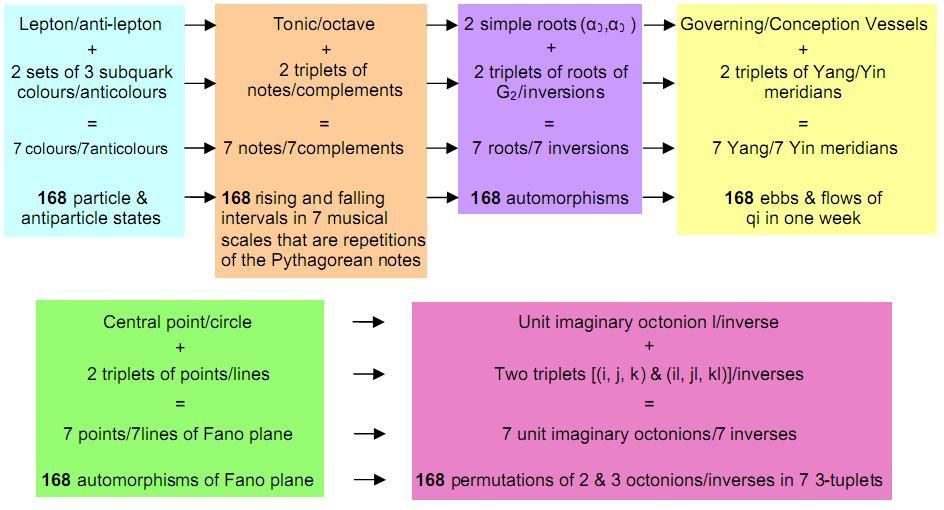Every two hours, the vital energy that Chinese acupuncturists call ‘qi’ ebbs and flows in

5

successive members of the 12 meridians other than the Conception and Governing Vessels. In one week, the 12 meridians undergo (7×12×2=168) ebbs and flows. The 24 ebbs and flows in one day correspond to the 24 particles of a given colour. The weekly cycle of seven days corresponds to the seven colour states of particles, the seven notes in the Pythagorean scale above the tonic, the seven roots of G2, the seven Yang or Yin meridians, the seven points or the seven lines of the Fano plane and the seven unit imaginary octonions ei (i = 1-7).

These holistic systems manifest a fundamental duality:

 7 colour states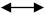7 anti-colour states 7 musical notes7 complements of notes 7 roots of G27 inversions of roots 7 Yang meridians7 Yin meridians 7 points of Fano plane7 lines 7 unit imaginary octonions (ei)7 inverses (ei–1 = -ei)

Each holistic system is invariant with respect to interchange of the members of each class of polar opposites. This duality is represented in the inner form of the Tree of Life as the two sets of seven regular polygons, one set being the mirror image of the other.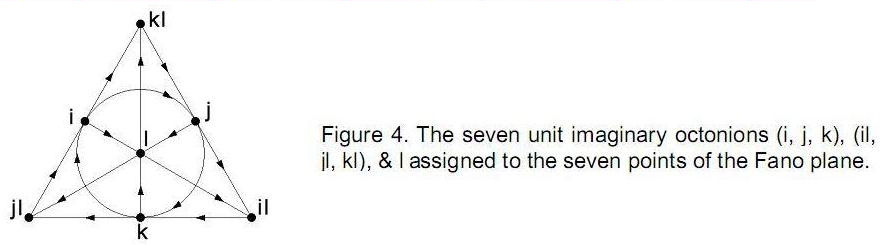Each septet of members of the holistic system comprises a singlet and two triplets:

7 colour states = colour singlet (1), colour triplet (3) + colour triplet (3').
7 musical notes = tonic (1) + (T, T2, T3) + (T2L2, T3L2, T4L2)                                                                                     (T = 9/8 & L = 256/243)
7 roots of G2 = α0 + (α1, α2, α3) + (α4, α5, α6).
7 meridians = Governing or Conception Vessel + 3 hand meridians + 3 foot meridians.
7 points of Fano plane = central point + triplet of points on circle + triplet of points at vertices of triangle (Fig. 4).
7 unit imaginary octonions = I + (i, j, k) + (il, jl, kl) (Fig.4).

This 1:3:3 pattern reflects how the seven Sephiroth of Construction comprise Malkuth and the two triads: Chesed-Geburah-Tiphareth & Netzach-Hod-Yesod.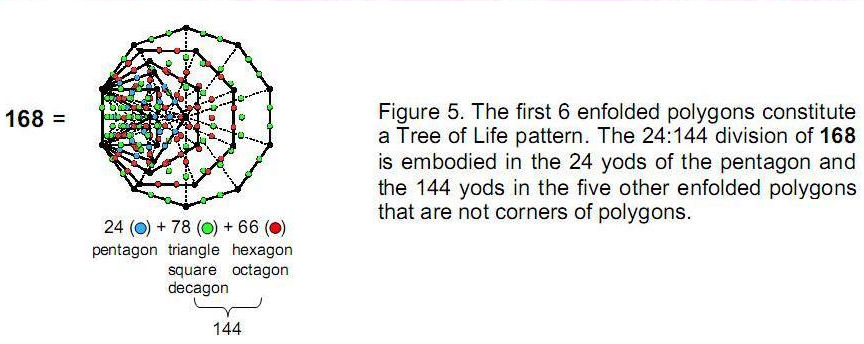6

The pattern of 24 leptons and 144 subquarks appears in the first six enfolded polygons (Fig. 5). Associated with them are 168 yods other than corners. Of these, 24 yods belong to the pentagon, the five other polygons having 144 yods. The number value 78 of Cholem is the number of yods in the triangle, square & decagon. The number value 90 of Yesodoth is the number of yods in the pentagon, hexagon & octagon.

The 24:144 division of the number 168 manifests not only in the first six enfolded polygons but also in both sets of these polygons. 168 yods lie outside their shared root edge on their 60 edges (Fig. 6). They include 24 corners of each set of polygons. There are 24 corners of one set of polygons (red yods) and 144 other (black) yods.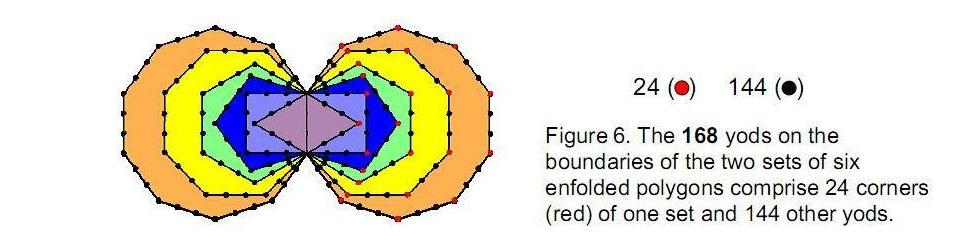The same 24:144 division manifests in the pair of Type B dodecagons. 120 vertices, edges & triangles surround the centre of each polygon. The 240 geometrical elements surrounding the centres of the two dodecagons consist of 72 triangles and 168 vertices & edges (Fig. 7). The latter comprise 24 corners of the dodecagons and 144 vertices & edges. The number 168 measures the number of geometrical elements that shape the 72 tetractyses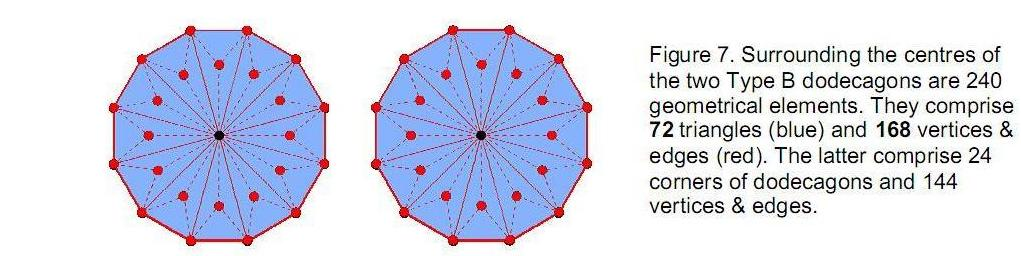making up the two Type B dodecagons.

The 24:144 division is also exhibited in the disdyakis triacontahedron (Fig. 8). Surrounding a vertical axis joining two diametrically opposite A vertices are 60 vertices. They comprise 24 vertices above the equator with 12 vertices and 24 vertices below it. 84 edges and 60 triangles (144 geometrical elements) are above and below the equator. The 168 geometrical elements composing each half of the disdyakis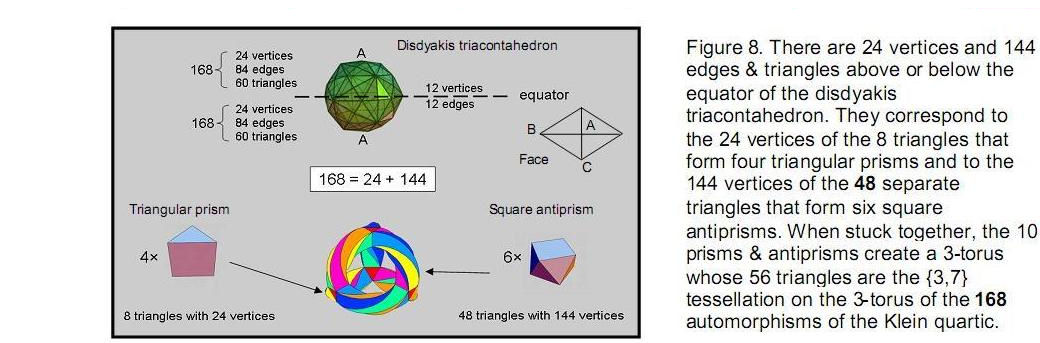7

triacontahedron above and below its equator are made up of 24 0-dimensional elements and 144 1- & 2-dimensional elements.

Fig. 8 also shows the {3,7} tessellation onto the 3-torus of the 56 orbits of order 3 that correspond to the 56 bitangent points of the Klein quartic.2 The 3-torus is topologically equivalent to six square antiprisms with their 12 square faces stuck to the 12 square faces of four triangular prisms in a tetrahedral formation, each antiprism being given a twist. This leaves 56 triangular faces, which, as separate triangles, have 168 vertices (eight from the triangular prisms with 24 vertices, 48 from the square antiprisms with 144 vertices). Each triangular prism has nine edges and each square antiprism has 16 edges, of which eight edges coincide with edges of the former when they are stuck together. This leaves (4×9 + 6×8 = 84) edges. The 84 edges of the 56 triangles in the tetrahedron and the 84 edges of its version turned inside-out correspond to the 84 edges above the equator of the disdyakis triacontahedron and their 84 mirror images.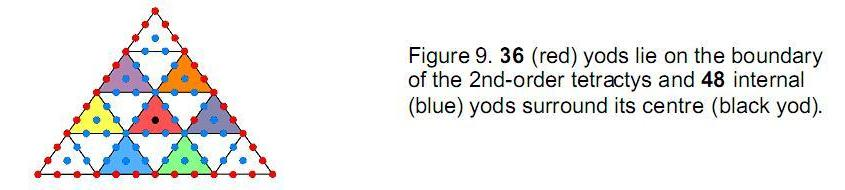The four triangular prisms at the apices of the tetrahedron have all their (4×9=36) edges joined to edges of the six twisted, square antiprisms forming its edges. The latter have (6×8=48) edges that are not joined. This 36:48 division of shape-forming edges has its counterpart in the 2nd-order tetractys, which is the abstract representation of holistic systems (Fig. 9). 36 (red) yods lie on its boundary and 48 (blue) yods are inside it surrounding its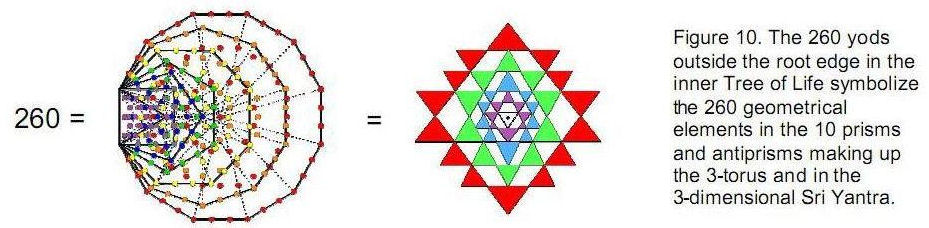centre (black yod). The 84 degrees of freedom symbolized by these yods characterize holistic systems. The 3-torus is revealed by its construction from four triangular prisms and six square antiprisms to be a holistic system because their edges possess the same 36:48 pattern displayed by the 2nd-order tetractys.

4. {3,7} tessellation of 3-torus with tetractyses

Consider the triangular faces of the triangular prisms and the square antiprisms making up the 3-torus, firstly, turned into tetractyses and, secondly, divided into their three sectors and the latter then turned into tetractyses. We shall calculate the numbers of yods in each case, noting that the 12 squares of the prisms become stuck to the 12 squares of the antiprisms, so that they play no part in the properties of the surface of the 3-torus. Their properties are listed below:

A triangular prism has 6 vertices, 9 edges, 2 triangles & 3 squares.
A square antiprism has 8 vertices, 16 edges (8 unshared), 8 triangles & 2 squares.

8

Table 2. Yod composition of the four triangular prisms & the six square antiprisms.

 Tetractys Three tetractyses Triangular Prism Square Antiprism Total Triangular Prism Square Antiprism Total Number of vertices 4×6 = 24 6×8 = 48 72 24 + 4×2 = 32 48 + 48×1 = 96 128 Number of edges 4×9 = 36 6×16 = 96 132 36 + 8×3 = 60 96 + 48×3 = 240 300 Number of triangles 4×2 = 8 6×8 = 48 56 8×3 = 24 48×3 = 144 168 Number of geometrical elements 68 192 260 116 480 596 Number of hexagonal yods on edges of tetractyses 2×36 = 72 2×96 = 192 264 2×60 = 120 2×240 = 480 600 Number of hexagonal yods 8 + 72 = 80 48 + 192 = 240 320 24 + 120 = 144 144 + 480 = 624 768 Number of yods on edges of tetractyses 24 + 72 = 96 48 + 192 = 240 336 32 + 120 = 152 96 + 480 = 576 728 Number of yods 8 + 96 = 104 48 + 240 = 288 392 24 + 152 = 176 144 + 576 = 720 896

The four triangular prisms have 24 vertices and the six square antiprisms have 48 vertices. The ten separate prisms and antiprisms have 72 vertices. This is the 36th even integer, showing how ELOHA, Godname of Geburah with number value 36, prescribes the geometry of the 3-torus. They have 132 edges, where 132 is the 65th even integer after 2. This shows how ADONAI, Godname of Malkuth with number value 65, prescribes the form of the 10 prisms and antiprisms through their edges.

A triangular prism has six vertices, nine edges and two triangles. A square antiprism has eight vertices, 16 edges and eight triangles. The two structural units are composed of 49 geometrical elements prescribed by EL ChAI, Godname of Yesod with number value 49. The four triangular prisms have 68 geometrical elements and the six square antiprisms have 192 geometrical elements. The ten separate prisms and antiprisms have 260 (=26×10)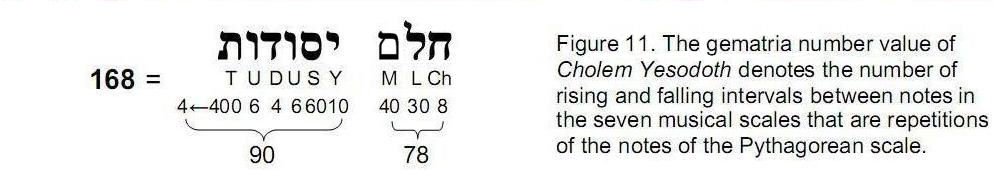geometrical elements. This demonstrates the holistic nature of the tessellated 3-torus that they form, for it is not only prescribed by YAHWEH with number value 26 but these elements are symbolized by the 260 yods outside the root edge of the seven enfolded polygons making up the inner form of the Tree of Life (Fig. 10). The 3-dimensional Sri Yantra is also composed of 260 geometrical elements.3

The 84 repeated, rising, Pythagorean intervals4 between notes of the seven musical scales have the composition: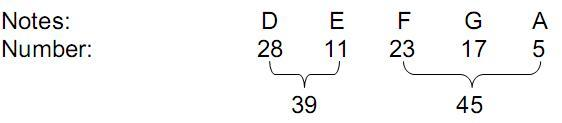Similarly for the 84 repeated, falling intervals. There are therefore (2×39=78) rising and falling major seconds and thirds and (2×45=90) rising and falling intervals of perfect fourths, perfect fifths & major sixths. Remarkably, 78 is the gematria number value of Cholem and 90 is the number value of Yesodoth, the two Hebrew words making up the Mundane Chakra of Malkuth (see Table 1) with number value 168 (Fig. 11). There are also 39 repeated intervals of a major third (E), perfect fourth (F) & major sixth (A), and

9

45 intervals of a major second (D) or perfect fifth (F). However, the former choice seems the correct one, intuitively speaking, because the two subsets follow the natural order of consecutive tone ratios of the notes D, E, F, and G & A.

Table 2 indicates that 264 hexagonal yods lie on the 132 edges of the 56 triangles of the 10 prisms and antiprisms. This confirms the Tree of Life character of the 3-torus formed from these building blocks because the seven enfolded polygons that constitute the inner form of the Treeof Life have 264 yods when their 47 sectors are turned into tetractyses (Fig. 12). Alternatively, the pair of 3-tori has 264 edges.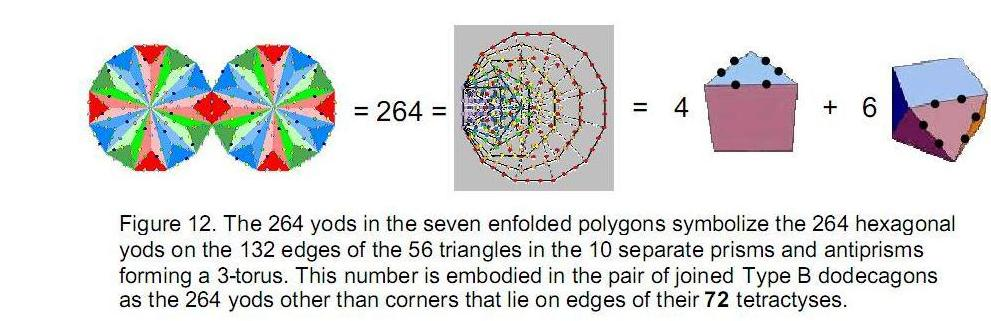Furthermore, there are 264 yods other than corners needed to delineate the edges of the 72 tetractyses in the pair of joined dodecagons when their sectors are each divided into three tetractyses.

According to Table 2, 336 yods lie on the edges of the 56 tetractyses of the 10 prisms and antiprisms. It is further confirmation of the Tree of Life nature of the {3, 7} tessellation of the 3-torus because this number has been discussed in many previous articles as a structural parameter of the E8×E8 heterotic superstring. It is the order of SL(2,7), the group describing the 336 automorphisms and anti-automorphisms of the Klein quartic whose Riemann surface is topologically equivalent to a 3-torus. There are 336 yods on the edges of the 42 triangles of the Sri Yantra, 336 yods other than corners in the two sets of the first six enfolded polygons and 336 yods other than corners in the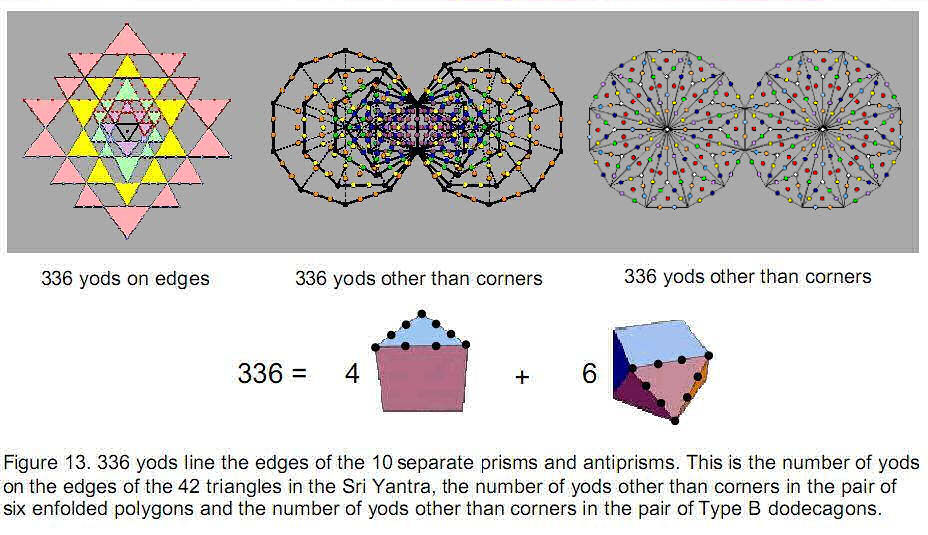10

pair of joined dodecagons when each sector is divided into three tetractyses (Fig. 13).

According to Table 2, the 168 tetractyses in the 10 separate prisms and antiprisms comprise 428 vertices & edges. 428 is the number value of Chasmalim (“Shining Ones”), the order of angels assigned to Chesed. They also contain 896 yods. Of these, 56 yods are centres of the 56 triangles. Hence, there are (896–56=840) yods other than these centres. In the case of all triangular faces being tetractyses, there are 336 yods other than these same centres delineating their edges. This is remarkable, for, as many previous articles have discussed, both the numbers 840 and 336 are structural parameters of the heterotic superstring. Each of its ten closed curves, or “whorls,”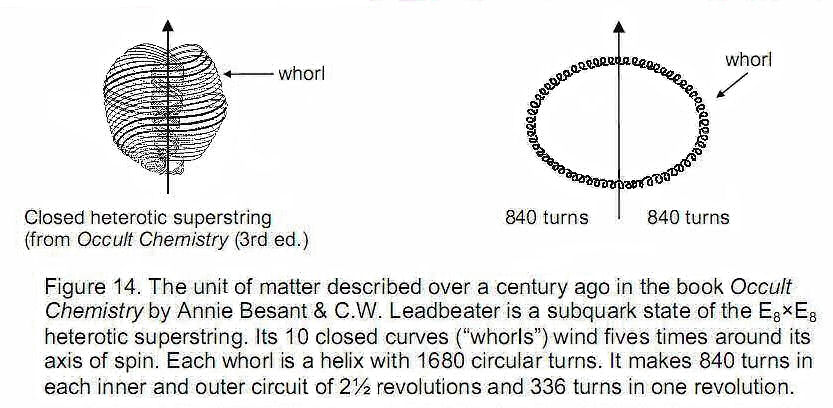winds 1680 times around a torus as it twists five times around its spin axis (Fig. 14). Each revolution makes 336 circular turns and one-half of a revolution makes 840 turns. If the 168 tetractyses making up the prismatic version of the 3-torus were separated from one another, they would have 1680 yods. This is the number of turns in each whorl. It is remarkable that the ten prisms and antiprisms embody three structural parameters of the unit of physical matter described over a century ago by Besant and Leadbeater.5 This cannot be coincidence. Instead, it indicates a basic role for the 3-torus in the underlying physics of superstrings as the microscopic manifestation of holistic systems, for each yod in its 168 tetractyses surrounding the centres of the 56 triangles denotes a turn of a whorl.

Let us now consider the prisms and antiprisms joined together at their square faces to form the 3-torus. Table 3 displays their geometrical and yod composition.

Table 3. Yod composition of the 3-torus constructed from tetractyses.

 Tetractys Three tetractyses Triangular Prism Square Antiprism Total Triangular Prism Square Antiprism Total Number of vertices 4×6 = 24 – 24 24 + 4×2 =32 48×1 = 48 80 Number of edges 4×9 = 36 6×8 = 48 84 36 + 8×3 = 60 48 + 48×3 = 192 252 Number of triangles 4×2 = 8 6×8 = 48 56 8×3 = 24 48×3 = 144 168 Number of geometrical elements 68 96 164 116 384 500 Number of hexagonal yods on edges of tetractyses 2×36 = 72 2×48 = 96 168 2×60 = 120 2×192 = 384 504 Number of hexagonal yods 8 + 72 = 80 48 + 96 = 144 224 24 + 120 = 144 144 + 384 = 528 672 Number of yods on edges of tetractyses 24 + 72 = 96 96 192 32 + 120 = 152 48 + 384 = 432 584 Number of yods 8 + 96 = 104 48 + 96 = 144 248 24 + 152 = 176 144 + 432 = 576 752

11

With single tetractyses as their faces, the four triangular prisms and the six square antiprisms are composed of 56 tetractyses with 248 yods. This remarkable, for 248 is the number of gauge bosons that transmit the unified force between superstrings of ordinary matter. Furthermore, the 248 yods comprise 8 yods at centres of the faces of the four triangular prisms, leaving 240 yods made up of 24 yods at vertices, 48 yods at centres of tetractyses, that is,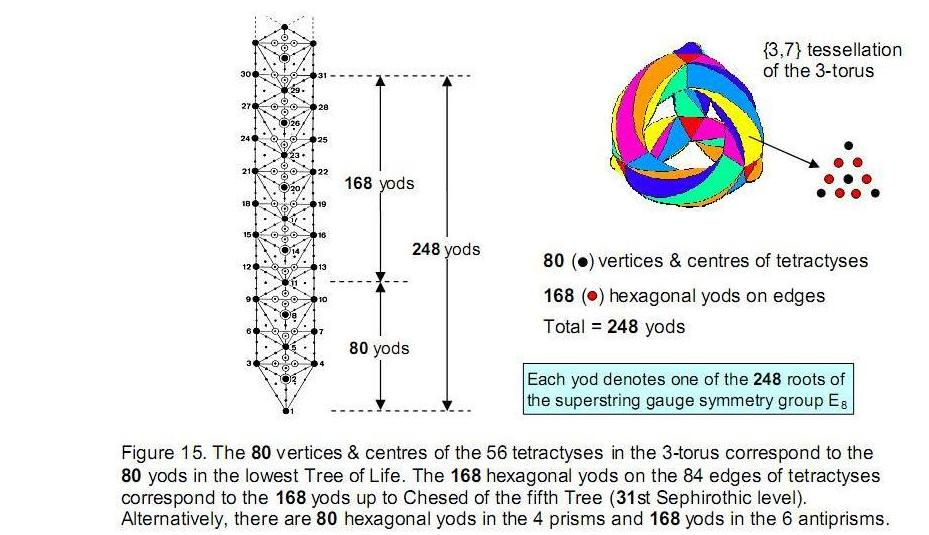72 yods that either vertices or centres of tetractyses, and 168 hexagonal yods on their 84 edges. The division:

248 = 8 + 240

corresponds to the eight simple roots of E8 and its 240 roots. The division:

240 = 72 + 168

corresponds to the 72 roots of E6, an exceptional subgroup of E8, and the 168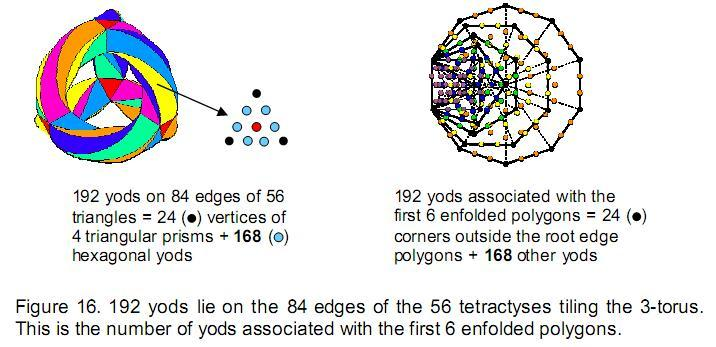12

remaining roots of E8. The root structure of the superstring gauge symmetry group E8 is embodied in the yod composition of the tessellation of the 3-torus in terms of tetractyses. As so often found in discussions of holistic systems, the number 168 defines the shape of the holistic system as — in the case of faces as single tetractyses — the number of hexagonal yods on the boundary of the tetractyses that compose it and — in the case of faces as three tetractyses — the number of triangles.

The 56 tetractyses in the 3-torus have 80 vertices and centres. Their 84 edges have 168 hexagonal yods. This 80:168 division appears in the lowest five overlapping Trees of Life constructed from tetractyses as the 80 yods of the lowest Tree and the 168 yods beyond it to Chesed of the fifth Tree — the 31st Sephirothic level prescribed by EL, the Godname of Chesed with number value 31 (Fig. 15).

According to Table 3, 192 yods lie on the 84 edges of the 56 tetractyses. 24 yods are vertices of the four triangular prisms. This 24:168 division corresponds in the inner form of the Tree of Life to the 24 external corners and to the 168 yods other than corners associated with each set of the first six enfolded polygons (Fig. 16). One set of polygons corresponds to a 3-torus. Its mirror image corresponds to the 3-torus turned inside out.

With faces of the prisms and antiprisms constructed from three tetractyses, the 3-torus is composed of 168 tetractyses with 80 vertices. 80 is the number value of Yesod. The four prisms with single tetractyses as their triangular faces have 80 hexagonal yods. The four triangular prisms have 32 vertices and the six square antiprisms have 48 vertices. This 48:32 division manifests in the 80 yods of the lowest Tree of Life as the 48 yods up to Chesed and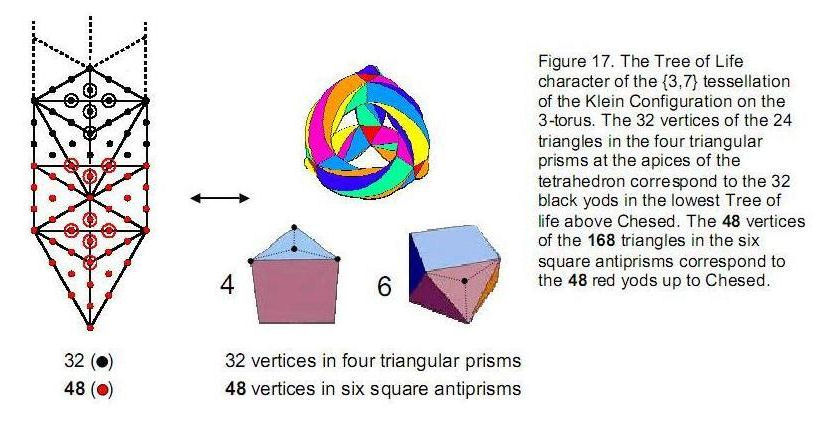the 32 yods above it (Fig. 17). The correspondence between the yods and geometrical elements demonstrates the Tree of Life character of the 3-torus.

The 168 tetractyses comprise 24 tetractyses in the four triangular prisms and 144 tetractyses in the six square antiprisms. The same 24:144 pattern is displayed by the first six enfolded polygons and by the dodecagon (see Figs 6 & 7). According to Table 2, the 3-torus has 248 vertices & triangles. With single tetractyses as faces, it has 80 vertices & triangles. Hence, 168 vertices & triangles are added by turning each face into three tetractyses. 168 edges are added as well, i.e., 336 geometrical elements are added. This 168:168 division of added elements reflects the 168 automorphisms and 168 anti-automorphisms of SL(2,7). The 3-torus with single tetractyses as faces has 168

13

hexagonal yods on the 84 edges of 56 tetractyses. With its triangles turned into three tetractyses, it has 504 hexagonal yods on the 252 edges of 168 tetractyses. 336 hexagonal yods are added. The counterpart in the Sri Yantra of this 168:336 division of the 504 hexagonal yods is the 168 geometrical elements in the fourth layer of 14 triangles and the 336 elements in the first three layers (see Table 3 of Article 35).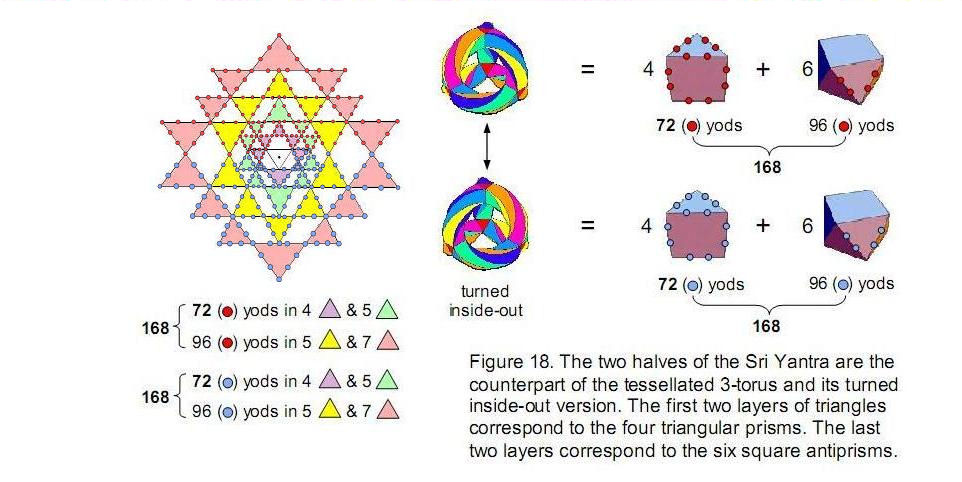The 3-torus is constructed from 500 (=50×10) geometrical elements (see Table 3). It is a remarkable demonstration of how ELOHIM, the Godname of Binah with number value 50, prescribes the geometry of the 3-torus constructed from triangles. We found earlier that the separate prisms and antiprisms have 260 (=26×10) geometrical elements when their triangular faces are regarded as single triangles. This is unmistakable evidence that YAHWEH and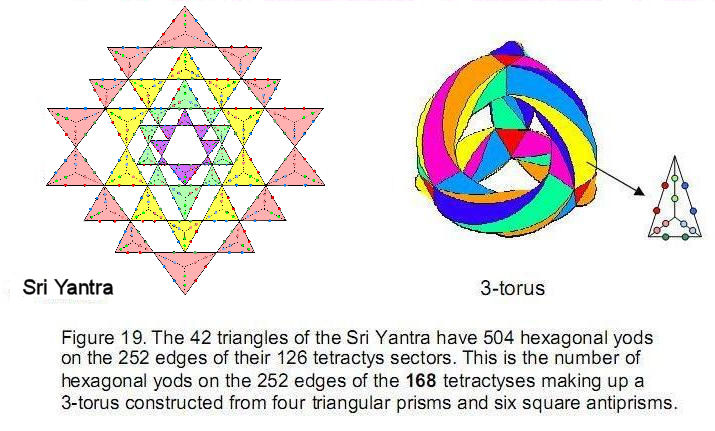ELOHIM prescribe the geometrical composition of the {3,7} tessellation of the 3-torus. The Klein Configuration embodies the divine archetypes.

14

According to Table 3, the four triangular prisms have 72 hexagonal yods on the 36 edges of their eight tetractyses and the six square antiprisms have 96 hexagonal yods on the 48 edges of their 48 tetractyses that are unshared with the former. This 72:96 division of the 168 hexagonal yods has its counterpart in the Sri Yantra (Fig. 18). When its 42 triangles are turned into tetractyses, 168 yods line the 63 edges of the 21 triangles in each half. The first two layers of 18 triangles have 144 yods on their 54 sides, 72 yods in each half. The last two layers of 24 triangles have 192 yods on their 72 sides, 96 yods in each half. The 168 boundary yods in each half comprise 72 yods in the first two layers and 96 yods in the last two layers. The former corresponds to the four triangular prisms and the latter correspond to the six square antiprisms. The 3-torus and its turned inside-out version are the counterpart of each half of the Sri Yantra.

Table 3 indicates that 504 hexagonal yods lie on the 252 edges of the 168 tetractyses. For single tetractyses as faces, there are 168 such yods. Hence, 336 (=2×168) hexagonal yods are added by the construction of each triangular face from three tetractyses. Remarkably, when the 42 triangles of the Sri Yantra surrounding the central one are each divided into their three sectors, the 126 sectors also have 252 edges with 504 hexagonal yods on them (Fig. 19). With their triangular faces divided into three tetractyses, 48 hexagonal yods are added to the triangular prisms and 288 hexagonal yods are added to the square antiprisms. Conversion of each triangle of the Sri Yantra into three tetractyses adds six hexagonal yods on edges. Compare the numbers of this type of yod for the two types of prism (red numbers denote number of hexagonal yods on edges inside the 56 primary triangles):

 4 triangular prisms 6 square antiprism 120 = 72 + 48 384 = 96 + 288

with the number of hexagonal yods on the edges of the tetractyses making up the four layers of triangles of the 3-dimensional Sri Yantra:

 8 triangles 10 triangles 10 triangles 14 triangles 96 120 120 168

The (72+96=168) hexagonal yods in the 3-torus with tetractyses as the triangles correspond to the 168 hexagonal yods on the 84 edges of the 42 tetractyses in the last set of 14 triangles. The (48+288=336) hexagonal yods added by the conversion correspond to the (96+120+120=336) hexagonal yods on the 168 edges of the 84 tetractyses in the first three sets of triangles. We see that the fourth set of triangles corresponds to the original 3-torus and that the first three sets of triangles correspond to the change in the 3-torus when its 56 triangles are each divided into three tetractyses. Moreover, the 42 sectors of the last set of 14 triangles have 84 edges, whilst the 84 sectors of the first three sets of triangles have 168 edges. This compares with the 84 edges of the 56 triangles of the 3-torus and the 168 edges added by dividing each of these triangles into its three sectors. The correspondence exists for the edges as well. The 3-torus embodies the properties of the Sri Yantra because it is a holistic system.

The number of yods making up the 168 tetractyses in the 3-torus is 752. This is 16×47, where 16 is the 15th integer after 1 and 47 is the 15th prime number. It shows how the Godname YAH with number value 15 prescribes the yod population of the 3-torus constructed from tetractyses. The Tetrad expresses the 176 yods in the four triangular prisms because 176 = 4×44. It expresses the 576 yods in the six square antiprisms because 576 = (1×2×3×4)2. It defines the 104 yods in the four triangular prisms with single tetractyses as its triangular faces because there are 26 combinations of objects arranged in the four rows of the tetractys and 104 = 4×26. It expresses

15

the 144 yods in the six square antiprisms with single tetractyses becauseWe found earlier that the 168 tetractyses making up the 10 separate prisms and antiprisms making up the 3-torus have 840 yods surrounding their centres. The counterpart of this property in the disdyakis triacontahedron (Fig. 20) are the 840 yods surrounding an axis that passes through two opposite vertices when its 120 faces are turned into tetractyses and when its 180 interior triangles with polyhedral edges as their edges are likewise transformed.6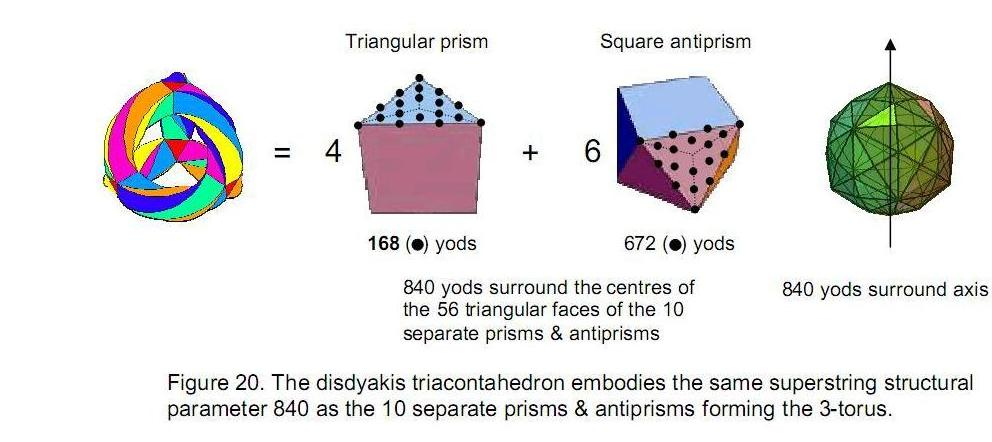Notice the ambient character of this number in both cases. The axis of the polyhedron is the counterpart of the centres of the 56 triangular faces of the 10 prisms and antiprisms. This is not coincidental, for the same character is displayed in the last regular polygon of the inner form of the Tree of Life (Fig. 21). When the 12 sectors of the dodecagon are transformed into 2nd-order tetractyses, one finds that there are 840 yods outside the root edge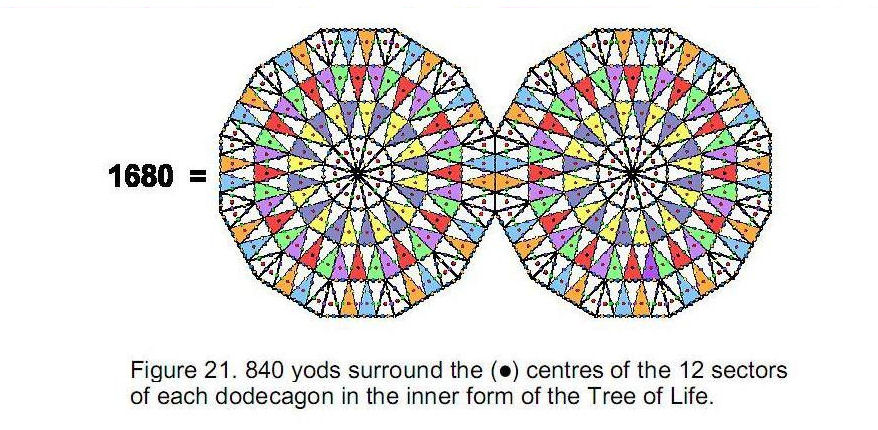surrounding their 12 centres. The 1680 yods surrounding the 24 centres in both dodecagons symbolize the 1680 turns of each whorl of the heterotic superstring (see Fig. 14). Each dodecagon corresponds to an inner or outer half of each curve with 840 helical turns and to the 3-torus

16

or to its turned-inside out version. These 24 centres remind us of the 24 vertices of the four triangular prisms forming the apices of the tetrahedron into which the 3-torus can be deformed. The lines joining these points are their 36 edges. Defining the shape of these prisms, the 24 points express the meaning of Malkuth, as symbolized by the 24 centres of the 2nd-order tetractyses in the dodecagon. The pair of mirror-image dodecagons is the inner Tree of Life counterpart of the 3-torus built from prisms and antiprisms and its turned inside-out version, similarly constructed.

5. Particle and musical counterparts of the {3,7} tessellation

A regular tessellation, or tiling, is a covering of the plane by regular polygons so that the same number of polygons meets at each vertex. The regular tessellations of the Euclidean plane are well-known. They are: {3,6} in which equilateral triangles meet six at each vertex; {4,4} in which squares meet four at each vertex; and {6,3} in which hexagons meet three at each vertex. A notation like {3,6} is called a Schläfli symbol. In general, {n,k} is the tessellation by polygons with n vertices, k polygons meeting at each vertex. If 1/n + 1/k < 0, {n,k} is a tessellation of the hyperbolic plane with negative curvature. If 1/n + 1/k = 0, {n,k} is a tessellation of the Euclidean plane with zero curvature. If 1/n + 1/k > 0, {n,k} is a tessellation of the elliptic plane with positive curvature. Poincaré described ways in which points in the hyperbolic plane can be represented conformally in the Euclidean plane. One of these is to represent the hyperbolic plane by points within the so-called ‘Poincaré disk’. {n,k} is the Poincaré dual of {k,n} in which vertices and polygons are interchanged.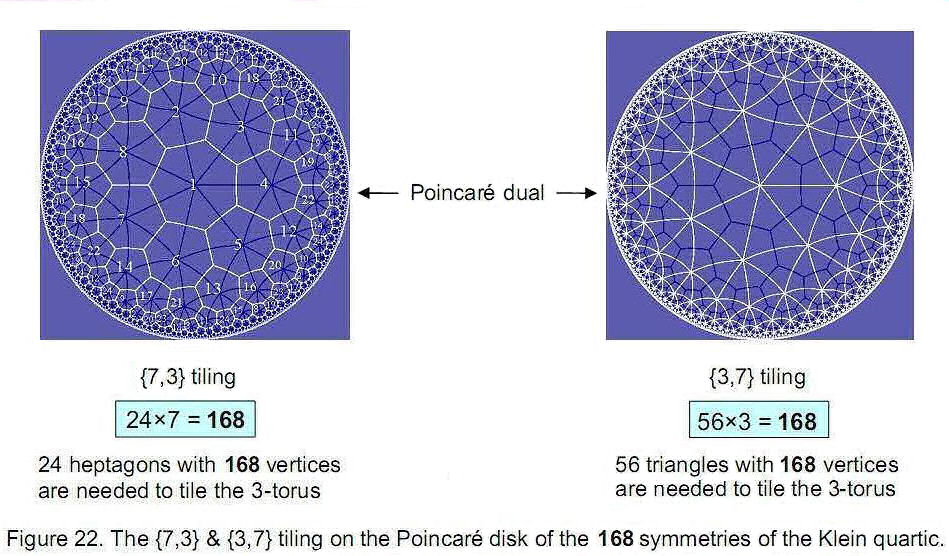SL(2,7) is the double cover of the Klein quartic symmetry group PSL(2,7). Its order 336 is the number of automorphisms and anti-automorphisms of the Klein quartic

x3 y + y3 z + z3 x = 0.

The 168 elements of PSL(2,7) are represented either by the {7,3} tiling of the Poincaré disk with 24 heptagons having 168 vertices or by its {3,7} tiling with 56 triangles having 168 vertices (Fig. 22).

17

The Riemann surface of the Klein quartic is a 168-sheeted covering of the sphere, branched over three points of the sphere.7

• Above one of these points, the 168 sheets join in groups of seven to give 24 points of the surface. These are the points of inflection of the curve described by the Klein quartic. They are also the Weirstrass points. The 24 orbits of subgroups of order 7 correspond to these points.
• Above another branch point, there are 84 points of the surface, where the sheets join in twos. These are the sextactic points through which pass a conic section that has six-fold contact with the curve. The 84 orbits of subgroups of order 2 correspond to them.
• Above the third branch point, the sheets join in threes to give 56 points of the surface. These 56 points are the points of contact of the Klein quartic with the 28 bitangents, or lines that are tangent to the curve at two points. The 56 orbits of subgroups of order 3 correspond to them.

PSL(2,7) has two families of seven groups of order 24, isomorphic to the octahedral group. This means that the 56 (=7×8) triangles of the 3-torus are associated with the eight vertices of seven cubes, each of whose 24 symmetries belong to the octahedral group; similarly for the turned-inside out version of the 3-torus, its 56 triangles define seven anticubes with 56 vertices.

In Article 40 (Part 4)8, a model of subatomic particles was presented that is analogous to the two groups of (7×24=168) symmetries. Three generations of basic particles, each in seven colour states, were hypothesized to exist. Each state further belongs to a group of eight consisting of four sets of two (matter/antimatter, weak isospin doublet & two supersymmetric partners). There are left-handed and right-handed versions of each particle, so that there are 168 left-handed particles and 168 right-handed particles. The two chiral families form seven groups of 24, each of the same colour. The following correspondence is established:

168 automorphisms/anti-automorphisms of Klein quartic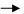168 left-/right-handed states;
24 orbits of subgroups of order 724 left-/right-handed states, each in 7 colours;
56 orbits of subgroups of order 356 left-/right-handed states, each in 3 generations;
84 orbits of subgroups of order 284 left-/right-handed states, each with its antimatter version.

The seven vertices of a heptagon in the {7,3} tiling of the Riemann surface of the Klein quartic are analogous to the seven colour states of basic particles, whilst the 24 heptagons correspond to the 24 particles of each colour. The three vertices of a triangle

Table 4. The tone ratios of the notes in eight octaves of the Pythagorean musical scale.

 C D E F G A B Number of overtones 1 1 9/8 81/64 4/3 3/2 27/16 243/128 0 2 2 9/4 81/32 8/3 3 27/8 243/64 2 3 4 9/2 81/16 16/3 6 27/4 243/32 4 4 8 9 81/8 32/3 12 27/2 243/16 7 5 16 18 81/4 64/3 24 27 243/8 11 6 32 36 81/2 128/3 48 54 243/4 15 7 64 72 81 256/3 96 108 243/2 20 8 128 144 162 512/3 192 216 243 26

18

in the {3,7} tiling denote the three generations of each particle, whilst the 56 triangles correspond to the 56 states of each generation.

If this analogy is not merely coincidental, it means that the gematria number value 168 (Fig. 11) of Cholem Yesodoth, the Mundane Chakra of Malkuth, the tenth Sephirah, denoting the universe of physical matter, measures not only the number of helical turns made by each whorl of the heterotic superstring as it revolves 180° around its spin axis but also the number of fermionic states in which the superstring exists. These states have 168 spin-0 supersymmetric partners (so-called ‘sfermions’).

Table 3 indicates that the 192 yods on the edges of the tetractyses making up the 3-torus are divided into their 24 vertices and the 168 hexagonal yods on their edges. Amazingly, this 24:168 division appears in the sequence of tone ratios of the notes of the Pythagorean scale shown in Table 4! The perfect fifth of the fifth octave has the tone ratio 24. It is the tenth overtone and the 32nd note above the tonic. There are 24 overtones up to the note G of the eighth octave with tone ratio 192, which is the 53rd note above the tonic. There are 14 overtones in 21 notes with a tone ratio difference of 168 between the 10th overtone with tone ratio 24 and the 24th overtone with tone ratio 192. Each yod on the boundaries of the prisms and antiprisms symbolizes a unit rise of pitch of a note of the Pythagorean scale. The 24 vertices of the four triangular prisms correspond to the first 24 harmonics up to the tenth overtone, their 72 hexagonal yods correspond to the next 72 harmonics up to 96, the 46th note above the tonic, and the 96 hexagonal yods on the edges of the six square antiprisms correspond to the final 96 harmonics up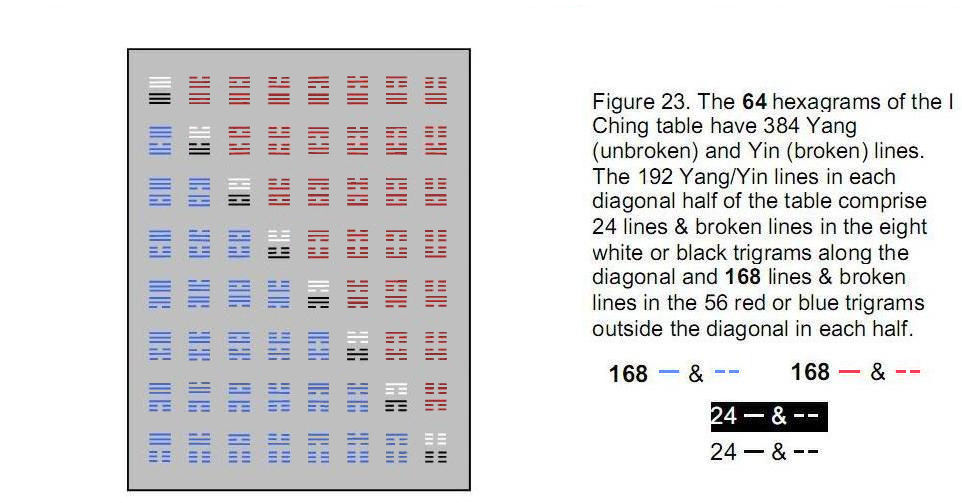to 192, the 24th overtone. There are also 168 harmonics up to this note, 24 of which are overtones of the Pythagorean scale, leaving 144 non-musical harmonics. This 24:144 division in the number 168 was encountered in the two sets of the first six enfolded polygons (Fig. 6), the pair of dodecagons (Fig. 7) and the disdyakis triacontahedron (Fig. 8) — all holistic structures embodying the divine archetypes. It occurs in the 192 lines and broken lines making up the 64 trigrams in each half of the I Ching table as the 24 Yang/Yin lines in the eight diagonal trigrams and the 168 Yang/Yin lines in the 56 off-diagonal trigrams (Fig. 23).

There are 384 harmonics up to the perfect fifth of the ninth octave. As this is the 61st note, all the Pythagorean notes up to this note can be assigned to the 61 yods in a decagon whose sectors are turned into tetractyses (Fig. 24). The 30 overtones can be assigned to the

19

30 yods on the boundary of the decagon and the 30 fractional tone ratios can be assigned to the 30 internal yods surrounding the central yod denoting the tonic 1. The system of 384 harmonics — the musical counterpart of the 384 yods in the pair of 3-tori — constitute a holistic system determined by the decagon, which is the symbol of the number 10, the Decad, called “All Perfect” by the Pythagoreans.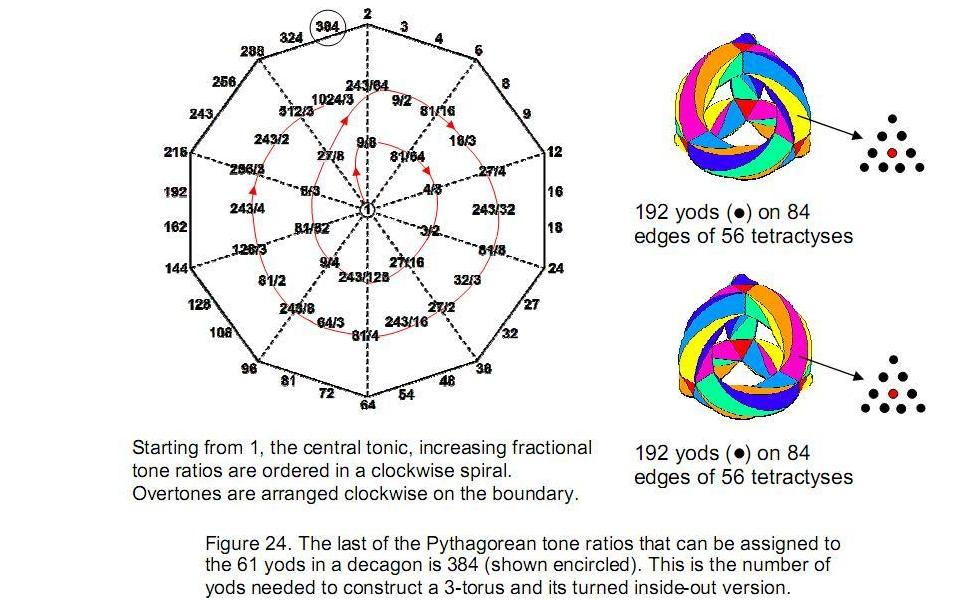As the eight notes of a musical scale have 27 intervals below the octave, the 44 notes of the seven musical scales have (7×27=189)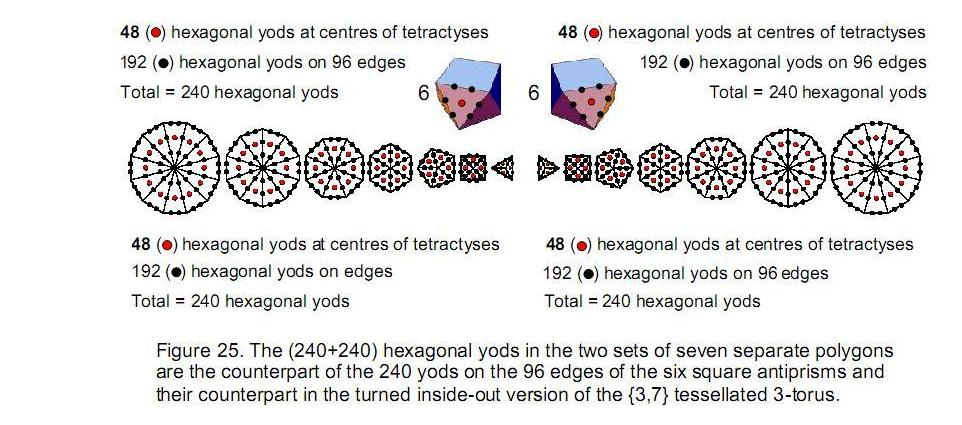20

intervals below the octave. They include 21 notes that are the second, third and fourth notes of each scale, leaving 168 intervals. Including the tonic, the octave and the unit interval between a note and itself, there are 192 intervals. They comprise 24 intervals (tonic, octave, unit interval and the 21 notes) and 168 intervals made up of the 21 complements of the 21 notes and 147 intervals between notes above the tonic (21 in each scale). We see that both the seven musical scales and the Pythagorean scale display the same 24:168 division found for the inner Tree of Life, the I Ching table, the Sri Yantra, the disdyakis triacontahedron, the 3-torus and the 192 harmonics up to the perfect fifth of the eighth octave.

The eight triangular faces of the four triangular prisms consist of eight sets of three vertices. They correspond to the eight upper trigrams in the diagonal of the I Ching table. The 168 hexagonal yods on the edges of the 10 prisms and antiprisms are arranged as 56 sets of two interlaced triangular arrays of three yods shared between adjoining tetractyses, that is, as 28 independent sets of two arrays. They correspond to the 28 hexagrams with 168 Yang/Yin lines above the diagonal. The (24+168=192) yods on the edges of the 56 tetractyses making up the turned-inside out version of the 3-torus corresponds to the 192 Yang/Yin lines in the lower, diagonal half of the table. The two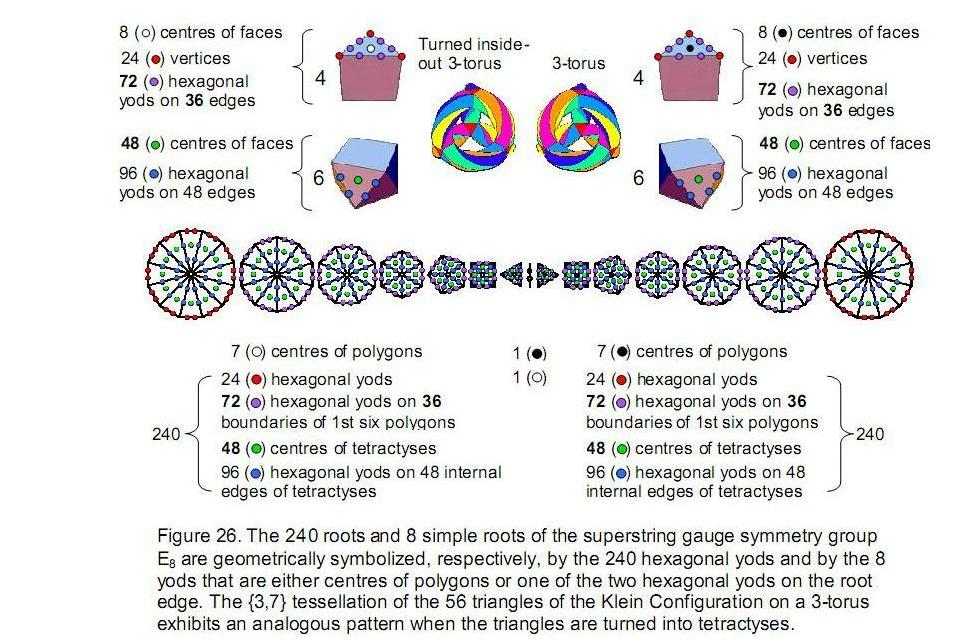diagonal halves of the I Ching table symbolize the 3-torus and its mirror image, just as the two sets of the first six enfolded polygons with (24+24) corners outside their root edge and (168+168) yods do.

According to Table 2, the 144 triangles of the six separate square antiprisms have 96 vertices and 240 edges when their 48 triangular faces are each divided into three triangles. There are 240 vertices & triangles. This 240:240 division displayed by their

21

geometrical composition has its counterpart in the two sets of seven separate polygons making up the inner form of the Tree of Life (Fig. 25). Each set has 240 hexagonal yods made up of 96 hexagonal yods on their edges and 144 hexagonal yods in their interiors. The 240 hexagonal yods in one set correspond to the 240 edges and the 240 hexagonal yods in the other set correspond to the 240 vertices and triangles. The 480 hexagonal yods signify geometrical degrees of freedom needed to construct the six arms of the 3-torus from points, lines and triangles. There are 480 hexagonal yods on the 240 edges of the 144 tetractyses making up the six separate square antiprisms. This property further demonstrates their Tree of Life basis.

In the context of superstring theory, the gauge symmetry group E8 has 240 roots and the symmetry group E8×E8 of heterotic superstrings has 480 roots. These (and the gauge bosons associated with them) are symbolized by the 240 pairs of hexagonal yods. The pairing of hexagonal yods on each edge of the 144 tetractyses reflects the direct product nature of the superstring symmetry group. It is evidence that E8×E8 heterotic superstrings, not SO(32) heterotic superstrings, are the basic constituents of matter.

With faces of the six antiprisms regarded as single tetractyses, Table 2 shows that they are made up of 240 hexagonal yods that comprise 48 hexagonal yods at the centres of the 48 tetractyses and 192 hexagonal yods on their 96 edges. They correspond to the 48 hexagonal yods at the centres of the 48 tetractyses in the seven separate polygons and their 192 hexagonal yods on the edges of these tetractyses (Fig. 26). The  two sets of polygons correspond to the 3-torus and its turned inside-out version. Figure 25 shows the detailed correspondence between the two sets of polygons and the two 3-tori. The eight yods consisting of the seven centres of polygons and one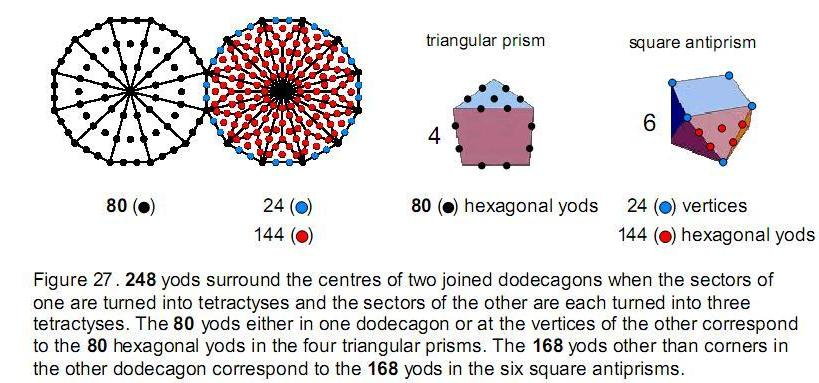of the two hexagonal yods in the separate root edge correspond to the yods at the centres of the eight triangular faces of the four triangular prisms. The 12 hexagonal yods lying on the boundary of the dodecagon are the counterpart of the 24 vertices of these prisms. The 72 hexagonal yods on the 36 edges of the first six polygons correspond to the 72 hexagonal yods on the 36 edges of these prisms. The hexagonal yods at the centres of the 48 tetractyses in the seven polygons correspond to the hexagonal yods at the centres of the 48 tetractyses in the faces of the six square antiprisms. The 96 hexagonal yods on the 48 interior edges of tetractyses of the seven polygons correspond to the 96 hexagonal yods on the 48 edges of these antiprisms. There is complete isomorphism

22

between the two geometries — one the representation in the Euclidean plane of the archetypal Tree of Life pattern and one its representation in the hyperbolic plane.

Suppose that the sectors of one dodecagon in the inner form of the Tree of Life are transformed into single tetractyses (Type A dodecagon) and that the sectors of the other one are each turned into three tetractyses (Type B dodecagon). 248 yods surround their centres (Fig. 27). As a holistic system, the pair of dodecagons embody the parameters of superstring physics — that is, the physical world of Malkuth. This is demonstrated by the fact that 280 yods outside the root edge other than yods at centres of tetractyses surround the centres of the two joined, Type B dodecagons. 280 is the number value of Sandalphon, Archangel of Malkuth. One Type B dodecagon has 168 yods other than corners surrounding its centre. 168 is the number value of Cholem Yesodoth, Mundane Chakra of Malkuth.

According to Table 3, the six square antiprisms have 168 yods. This is the number of yods other than its corners that surrounding the centre of the Type B dodecagon. The remaining 80 yods correspond to the 80 hexagonal yods in the four triangular prisms. The isomorphism found between the two tori and the two sets of seven separate polygons exists also for the two dodecagons when their sectors are turned into either one or three tetractyses. Furthermore, the distinction between the four ‘corners’ and the six ‘edges’ of the tetrahedron into which the 3-torus can be deformed defines the same 80:168 division of the number 248 found for the lowest five overlapping Trees of Life (Fig. 15) and for the 3-torus, which has 80 vertices and centres of tetractyses and 168 hexagonal yods on their edges, as well as 80 vertices and 168 tetractyses when the triangular faces are turned into three tetractyses.

According to Table 1, 80 is the number value of Yesod, which means “foundation.” Just as the lowest Tree of a set of overlapping Trees of Life has 80 yods and is their foundation, so the four triangular prisms with 80 hexagonal yods can be regarded as the foundation of the 3-torus because they are the ‘vertices,’ so to speak, of the tetrahedron into which the 3-torus can be deformed. According to Table 2, the ten separate prisms and antiprisms have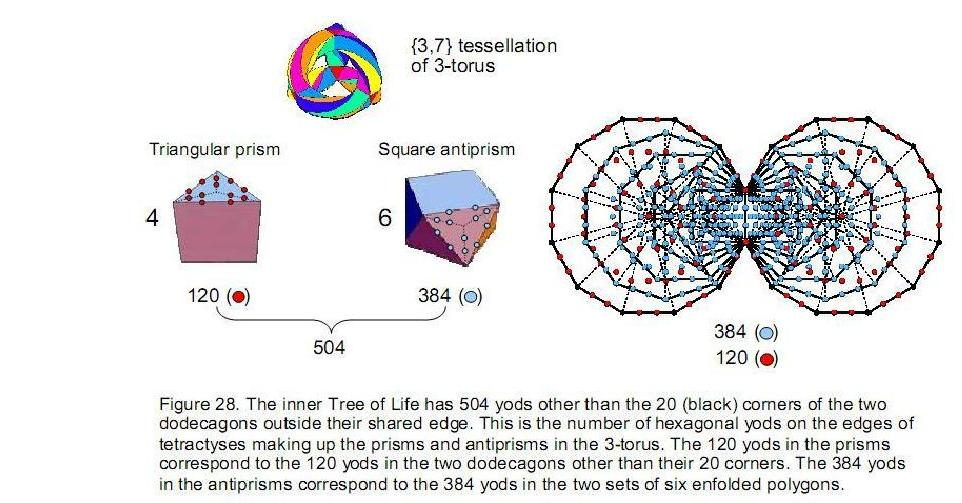(80+240=320) hexagonal yods. The 3-torus and its version turned inside-out have 640

23

hexagonal yods. 640 is the number value of Shemesh (“the Solar Light”), the Mundane Chakra of Tiphareth. The eight triangular prisms in the 3-torus and its turned-inside out version with single tetractyses as triangular faces have 48 vertices, whilst the six antiprisms have 48 faces. 48 is the number value of Kokab (“The Stellar Light”), the Mundane Chakra of Hod. With three tetractyses as their faces, they have 64 vertices. 64 is the number value of Nogah (“Glittering Splendour”), the Mundane Chakra of Netzach. The 3-torus and its turned inside version need 496 yods to construct their triangular faces from tetractyses. Table 3 indicates that the 3-torus with each face of a prism and antiprism divided into three triangles consists of 248 vertices and triangles. The tessellated 3-torus and its turned inside-out version have 496 vertices and edges, as well as 496 yods in the case where their faces are single tetractyses. 496 is the number value of Malkuth. They have 112 faces. 112 is the number value of Beni Elohim, the order of angels assigned to Hod.

Table 3 indicates that there are 584 yods on the edges of the 168 tetractyses making up the prisms and antiprisms. Suppose that these 168 triangles are divided into their 504 sectors and each sector turned into a tetractys. This adds 168 vertices to the 80 vertices already present, so that there are now 248 vertices — the same as the number of yods in the prisms and antiprisms assembled in the 3-torus when each of their faces is a tetractys. The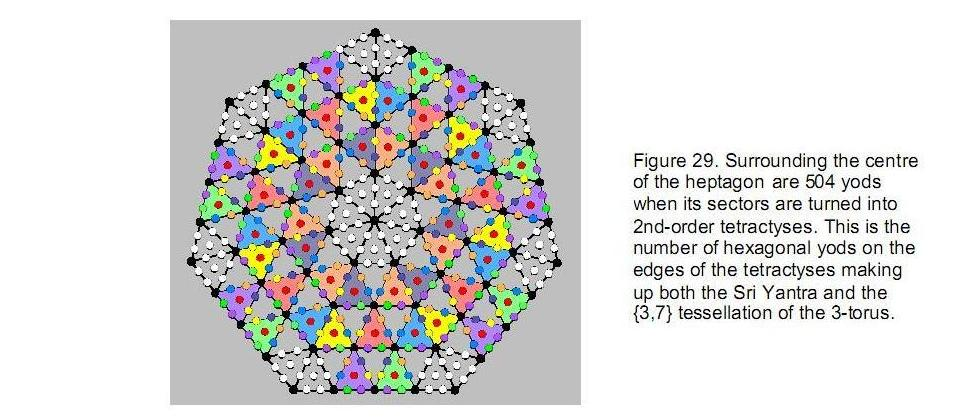conversion of each of the 168 triangles adds seven yods on edges of tetractyses. There are (7×168 + 584 = 1760) yods on the 756 edges of the 504 tetractyses. (1760–80=1680) yods on these edges surround the vertices and centres of the original 56 triangles used in the {3,7} tessellation of the 168 automorphisms of the Klein quartic. This higher-order conversion thus leads to the simultaneous appearance of the superstring structural parameter 1680 and the dimension 248 of the superstring gauge symmetry group E8 as properties of the {3,7} tessellation of the 3-torus. This cannot be due to chance. Instead, it confirms that the object described over a century ago by Annie Besant and C.W. Leadbeater as the basic constituent of matter is, indeed, a superstring.

The counterpart of this property in the disdyakis triacontahedron is the 1680 vertices, edges & triangles that surround its axis when the triangles in its interior formed by edges and its centre are each divided into three sectors.9

24

As discussed in Section 4, 504 hexagonal yods lie on the 252 edges of the 168 tetractyses formed when the 56 triangles in the {3,7} tessellation of the 3-torus are each divided into three tetractyses. This is the number of hexagonal yods lying on the 252 edges of the 126 tetractyses making up the Sri Yantra when each of the 42 triangles surrounding its central one is divided into three tetractyses (Fig. 19). It is further confirmation that this tessellation of the automorphisms of the Klein quartic is a manifestation of the mathematical archetypes embodied in the Sri Yantra, Tree of Life and all other examples of sacred geometry. This is also demonstrated by the fact that there are 504 yods in the inner form of the Tree of Life other than the 20 corners of the dodecagons outside their shared edge. That this is not coincidental is shown by the fact that the two dodecagons have 120 yods other than these corners and the two hexagonal yods in the shared edge, whilst the two sets of the first six polygons have 384 yods (Fig. 28). They correspond to the 120 hexagonal yods on the edges of the tetractyses making up the four triangular prisms and to the 384 hexagonal yods on the edges of the tetractyses making up the six square antiprisms. This 120:384 division is another Tree of Life pattern.

Table 3 shows that there are 248 yods in the tessellated 3-torus with single tetractyses as each triangle and 752 yods with three tetractyses as each triangle. 504 yods are added by the higher-order transformation. Nine hexagonal yods are added to each of the 56 primary triangles (9×56=504). They comprise 168 hexagonal yods at centres of the 168 tetractyses and 336 hexagonal yods on their 168 edges. In the Sri Yantra, the former correspond to the 168 hexagonal yods on edges of the 14 triangles in the fourth layer and the latter correspond to the 336 hexagonal yods on edges of the 28 triangles in the first three layers. Such correlation is strong evidence of the holistic nature of the {3,7} tessellation of the 3-torus and the relevance to superstring theory of the Klein quartic.

The heptagon has 504 yods surrounding its centre when its seven sectors are turned into 2nd-order tetractyses (Fig. 29). As illustrated earlier, all holistic systems are seven- fold in their physical manifestation. 43 yods — the number of triangles in the Sri Yantra — are needed to construct the heptagon from 1st-order-tetractyses. 504 yods are needed to construct it from 2nd-order tetractyses. Notice that the 70 tetractyses in this heptagon have 70 vertices surrounding its centre. The sum of the first 70 odd integers after 1 is 5040:

712 – 1 = 3 + 5 + 7 + … + 141 = 5040.

This is the number of yods in 504 tetractyses. If the 70 odd integers after 1 are assigned to the 70 vertices, we generate the same number as the number of yods obtained by replacing each of the 504 yods by tetractyses. There is always a harmony between number and sacred geometry. Figure 14 shows that three of the 10 whorls in the unit of matter described by Besant & Leadbeater are thicker than the seven other ones. As each whorl is a helix with 1680 turns, these three ‘major whorls’ have (3×1680=5040) helical turns. As the microscopic manifestation of the Tree of Life and tetractys paradigm, the three major whorls correspond to the yods at the corners of the tetractys, which symbolize in Kabbalah the Supernal Triad of Kether, Chokmah and Binah and in Hinduism the trimûrti of Shiva, Brahma and Vishnu — the triple Godhead.

The Cosmic Tree of Life10 maps all levels of reality, including the 26-dimensional space- time continuum. It consists of 91 overlapping Trees of Life with 550 Sephirothic levels (Fig. 30). The lowest seven Trees of Life with 47 Sephirothic levels map space-time. The 503 levels above the top of the seventh Tree span all superphysical levels of consciousness. Hence, the top of the seventh Tree marking the first of the 25 spatial

25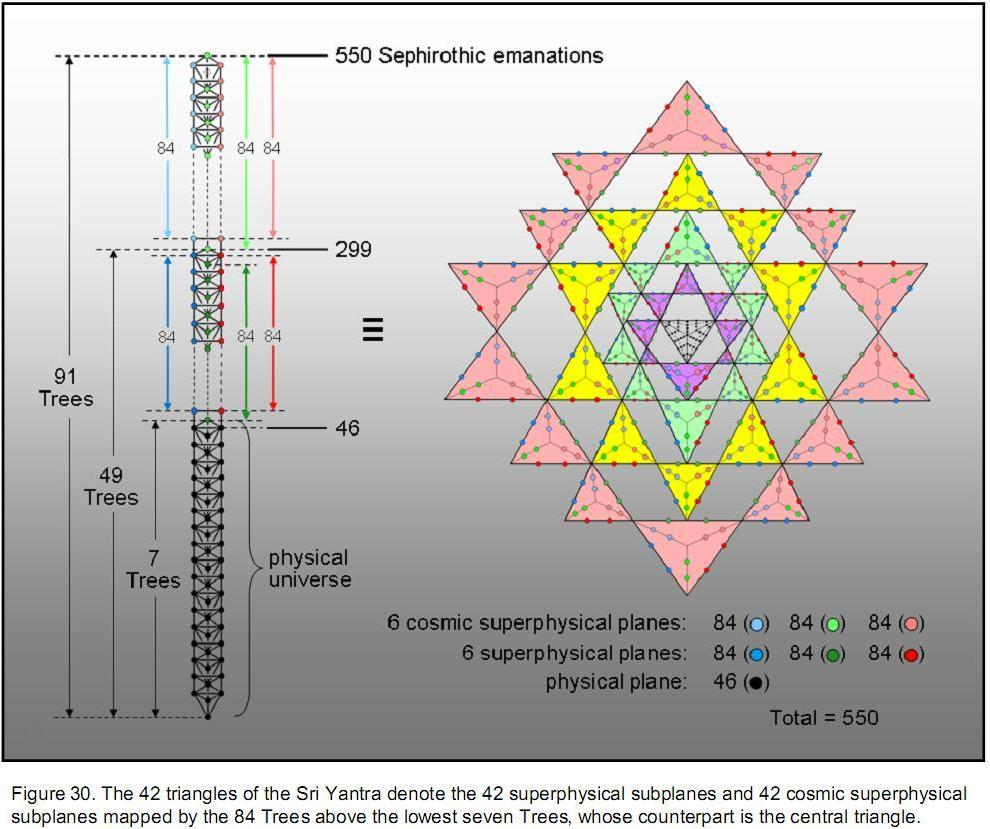26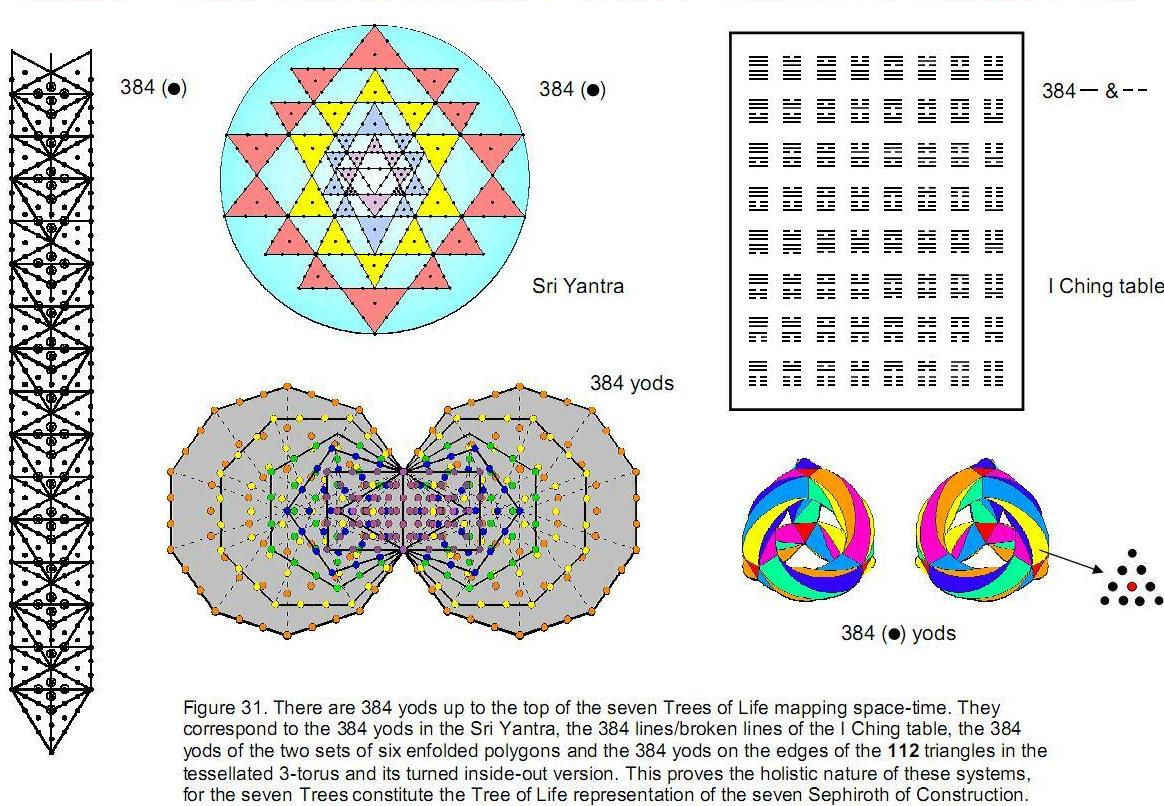27

dimensions11 is the 504th from the top of the 91st Tree. The 504 hexagonal yods in the 42 triangles of the Sri Yantra when each is divided into three tetractyses symbolise the 504 Sephirothic levels down to the top of the lowest seven Trees mapping space-time. The 252 yods on the internal edges of tetractyses symbolize the 252 Sephirothic levels down to the top of the 49th Tree that map the 42 subplanes of the six cosmic superphysical planes of consciousness. The 252 hexagonal yods on the sides of the 42 triangles symbolize the 252 Sephirothic levels from the top of the 49th Tree to the top of the seventh Tree that map the 42 subplanes of the six superphysical planes of consciousness. The final 46 Sephiroth levels of the seven lowest Trees are symbolized by the 46 yods of the central triangle, which signifies the physical universe/plane.

There are 84 Sephirothic emanations on each pillar of the Cosmic Tree of Life spanning the 42 cosmic superphysical subplanes. There are also 84 emanations on each pillar spanning the 42 cosmic physical subplanes above the physical plane. These (3+3=6) sets of 84 emanations correspond to the three exterior and three interior edges of the tetractyses in each triangle in the Sri Yantra. Yods on exterior edges correspond to the emanations in the 42 superphysical subplanes of the cosmic physical plane. Yods on interior edges correspond to the emanations in the 42 subplanes of the six cosmic superphysical planes. Intuitively speaking, it seems reasonable to associate the hexagonal yods in the base of each outward-pointing triangle with emanations of the central Pillar of Equilibrium and to associate hexagonal yods on their inclined edges with emanations on the side pillars. Similarly, it is reasonable to associate the hexagonal yods on the edges of tetractyses converging to the tip of each triangle with emanations of the uppermost 42 Trees on the central pillar, the hexagonal yods on inclined internal edges being associated with the emanations on the side pillars.

There is an alternative correlation between yods in the Sri Yantra and the emanations of the Cosmic Tree of Life. It is motivated by the fact that the 3-torus, when constructed from prisms and antiprisms, have 168 hexagonal yods on their 84 edges when their 56 triangular faces are tetractyses, and by the fact that a further 336 (=2×168) hexagonal yods are added on 168 more edges when these faces are each divided into three tetractyses. In other words, the 504 hexagonal yods on the 252 edges of 168 tetractyses bear the same 168:2×168 pattern that we see in the Cosmic Tree of Life for the 168 emanations on the central pillar down to the top of the seventh Tree and the 336 emanations above it on the two side pillars. This is no coincidence, for the {3,7} tessellation of the 168 automorphisms of the Klein quartic is a holistic system exhibiting the characteristics of the universal blueprint called the “Tree of Life.” A way of looking at the Sri Yantra that reproduces the 168:2×168 division in both the Cosmic Tree of Life and the tessellated 3-torus becomes apparent when we notice that the first three sets of triangles number 28 — twice the number in the fourth set. This means that the set of 14 triangles with 84 internal hexagonal yods and 84 external hexagonal yods corresponds, respectively, to the 84 emanations on the central pillar down to the top of the 49th Tree and to the next 84 emanations on this pillar down to the top of the seventh Tree. The 28 triangles have (6×28=168) hexagonal yods on their edges and 168 hexagonal yods on interior edges of tetractyses. These 336 yods correspond to the 168 emanations on each side pillar down to the top of the seventh Tree and to the 336 hexagonal yods added by turning each triangle of the tessellated 3-torus into three tetractyses. The 1:2 proportion of triangles in the four layers of the Sri Yantra is the counterpart of the division of the three pillars of the Cosmic Tree of Life into the central Pillar of Equilibrium and the two side pillars — the Pillar of Mercy and the Pillar of Severity.

We see that the 504 hexagonal yods on the edges of the 168 tetractyses making up the 56 hyperbolic triangles of the {3,7} tessellation of the 168 automorphisms of the Klein

28

quartic have a connotation that goes beyond the physics of superstrings — or even pure mathematics. This is because the properties of holistic systems have different, but analogous, levels of meaning. The 384 yods on the edges of the 112 triangles in the pair of 3-tori are the counterpart of the 384 yods up to the top of the seventh Tree mapping space-time (Fig. 31). The 48 vertices of the triangles correspond to the 48 yods up to Chesed of the lowest Tree. The correlation once again furnishes strong evidence that the {3,7} tiling of the 3-torus with 56 hyperbolic triangles constitutes a holistic system of fundamental significance to particle physics.

The nature of the three branch points of the 168-sheeted Riemann surface of the Klein quartic is analogous to the different ways of grouping the 168 Sephirothic emanations in each pillar of the 84 Trees of the Cosmic Tree of Life above the lowest seven Trees. The following correspondences exist:

1. The 84 sextactic points of the Klein quartic where the sheets join in twos correspond to the 84 pairs (×2) of Sephiroth on each pillar of the 84 Trees (Geburah-Binah, Tiphareth-Yesod or Chesed-Chokmah):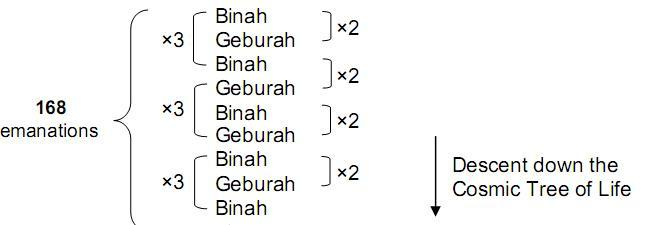The counterpart in the Sri Yantra of the 168 emanations on the Pillar of Equilibrium are the 84 pairs of hexagonal yods on external and internal edges of the 42 tetractyses composing the fourth layer of 14 triangles when each triangle is divided into three tetractyses (Fig. 30). The 42 external edges correspond to the 42 superphysical subplanes and the 42 internal edges correspond to the 42 cosmic superphysical subplanes. Similarly, the 84 pairs of hexagonal yods on external edges of the first three layers of 28 triangles correspond to the 84 pairs of emanations on the side pillars for the 42 superphysical subplanes, whilst the 84 pairs of hexagonal yods on internal edges of these triangles correspond to the 84 pairs of emanations on side pillars for the 42 cosmic superphysical subplanes. There have to be 14 triangles in the last layer because each side of a triangle corresponds to one of the 42 superphysical subplanes, and 42/3 = 14. The 336 hexagonal yods (168 pairs) on the internal edges of the 168 tetractyses making up the tessellated 3-torus correspond to the 336 emanations on the side pillars of the Cosmic Tree of Life above the seventh Tree, 168 emanations on each one. The 168 hexagonal yods on the 84 edges of the 56 triangles correspond to the 168 emanations on the central Pillar of Equilibrium down to the top of the seventh Tree;
2. The 56 points that are points of contact of the Klein quartic with the 28 bitangents correspond to the 56 triplets (×3) of Sephiroth on each side pillar (the Pillar of Judgement is used as the example shown above). Notice that there are 28 triplets of one combination of Sephiroth and 28 triplets of another combination. This corresponds to the 28 bitangents, each with two points of contact. Their counterpart is the {3,7} tessellation of the 3-torus into 56 triangles with 168 vertices. The counterparts of the 28 bitangents in the I Ching table are the 28 hexagrams on each
29

side of its diagonal (Fig. 23), the 168 lines and broken lines in one diagonal half denoting the 168 automorphisms of the Klein quartic and the 168 lines and broken lines in the other half denoting its 168 anti-automorphisms. The two points of contact of the Klein quartic with each bitangent are symbolized by the pair of different trigrams in each off-diagonal hexagram. In the Sri Yantra, their counterparts are the 28 sets of two groups of three hexagonal yods on external and internal edges of the 42 tetractyses in the 14 outermost triangles. There are 28 groups of three hexagonal yods on internal edges corresponding to cosmic superphysical subplanes and 28 groups of three hexagonal yods on external edges corresponding to superphysical planes. As with the Cosmic Tree of Life, the 168 yods form 56 triplets, 28 triplets of one type and 28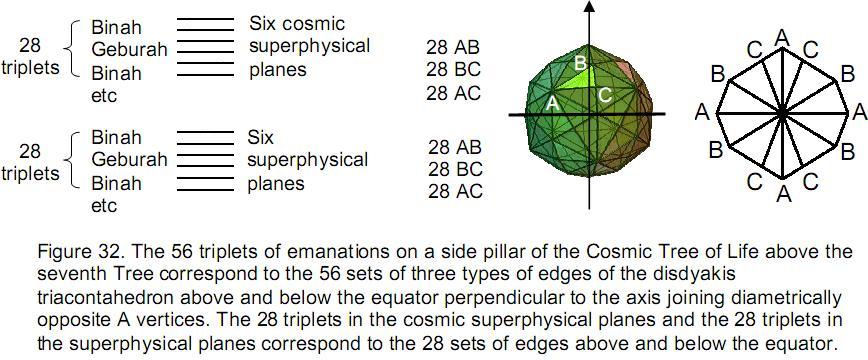of another type.

The counterpart in the disdyakis triacontahedron of the 168 sheets of the Riemann surface of the Klein quartic joining together in 56 sets of three is the following: the polyhedron (Fig. 32) has 180 edges made up of 56 sets of edges AB, BC & AC in the polyhedron above and below the equator formed by a 12-sided polygon with four AB edges, four BC edges & four AC edges. The 28 sets of three emanations on a side pillar of the Cosmic Tree of Life mapping the 42 subplanes of the six cosmic superphysical planes correspond in the polyhedron to the 28 sets of three edges above its equator. The 28 sets of three emanations on a side pillar for the 42 subplanes of the six superphysical planes correspond to the 28 sets of three edges below the equator;

1. The 168 sheets join in groups of seven to form the 24 Weirstrass points. The 84 Trees map the six cosmic superphysical planes, each of seven subplanes, and the six superphysical planes, each of seven subplanes. Each of the seven generic subplanes is repeated (6+6=12) times. A Tree of Life maps each subplane and there are two Sephiroth on the side pillar per Tree. The 168 emanations on a side pillar can be grouped into seven sets of (12×2=24), each set being associated with a Sephirah of Construction. The 24 Weirstrass points in each of the seven groups correspond therefore to the 24 emanations on a side pillar associated with each generic subplane, that is, with each Sephirah of Construction. For example, the third subplane of each plane (counting from the lowest subplane) corresponds to Hod, the third Sephirah of Construction from Malkuth. This Sephirah has 12 subplane manifestations, each mapped by a Tree of Life with two emanations on a side pillar associated with it, i.e., associated with Hod are 24 emanations. Similarly, 24 emanations in the Cosmic Tree of Life are associated with each of the six other Sephiroth of Construction. Their mathematical counterpart is the {7,3} tessellation of

30

the 3-torus as 24 heptagons, each with seven vertices. In the {7,3} tessellation on the 3-torus, the 56 triangular faces define the 56 vertices of seven cubes, each having the 24 symmetries of the octahedral cube. The 168 symmetries of the Klein quartic belong to PSL(2,7), which is of order 168. It has seven subgroups of order 24, isomorphic to the octahedral group. In terms of the model of particle physics presented in Part 4 of Article 40,12 each subgroup describes the 24 particles in each of the seven colour states. The clustering of the 168 sheets of the Riemann surface of the Klein quartic into 56 groups of three corresponds to the three generations of 56 types of particles.

The seven-fold nature of the 168 symmetries of the Klein quartic is the manifestation of the seven Sephiroth of Construction, which determine both the seven planes and the seven subplanes of each plane. The 168 automorphisms mapped on the 3-torus tessellated into 56 triangles correspond to the 168 emanations on one side of the Cosmic Tree of Life down to the top of the seventh Tree. The 168 anti-automorphisms mapped by the tessellated 3-torus turned inside out correspond to the 168 emanations on the opposite pillar, on which are located Sephiroth of opposite polarity. The positive and negative dualities embodied in the Pillars of Mercy and Judgement manifest in the projective duality of the 3-torus, which maps the 168 automorphisms, and its turned inside-out version, which maps its 168 anti-automorphisms. The two tori embody the 336 Sephirothic emanations of the side pillars down to the physical universe. Cholem Yesodoth, the Mundane Chakra of Malkuth, the Sephirah signifying this universe, has the number value 168 because it defines the location in the Cosmic Tree of Life of the physical plane mapped by its lowest seven Trees of Life as the number of emanations on the central Pillar of Equilibrium down to the top of the seventh Tree.

References

1 Phillips, Stephen M. Article 40 (Part 1): “The unification of all sacred geometries and its implication for particle physics,” (WEB, PDF), pp. 23-28.

2 Phillips, Stephen M. Article 15: “The mathematical connection between superstrings and their micro-psi description: a pointer towards M-theory,” (WEB, PDF), p. 24 et seq.

3 Phillips, Stephen M. Article 35: “The Tree of Life nature of the Sri Yantra and some of its scientific meanings,” (WEB, PDF), Table 1, pp. 2, 5.

4 Phillips, Stephen M. Article 16: “The tone intervals of the seven octave species and their correspondence with octonion algebra and superstrings,” (WEB, PDF), p.17.

5 Besant, Annie & Leadbeater, C.W. “Occult Chemistry,” 3rd ed., Theosophical Publishing House, Adyar, Madras, India, 1951.

6 Phillips, Stephen M. Article 27: “How the disdyakis triacontahedron embodies the structural parameter 1680 of the E8×E8 heterotic superstring,” (WEB, PDF), p. 19.

7 The Klein quartic is fully analysed in the book “The Eightfold Way: The Beauty of Klein’s Quartic Equation,” edited by Silvio Levy, Cambridge University Press, 1999. This is downloadable as PDF files from: http://www.msri.org/publications/books/Book35/index.html.

8 Ref. 1, Part 4, pp. 38-47.

9 Ref. 6, p. 6.

10 Phillips, Stephen M. Article 5: “The superstring as microcosm of the spiritual macrocosm,” (WEB, PDF), pp. 2, 3.

11 Phillips, Stephen M. Article 2: “The physical plane and its relation to the UPA/superstring and space- time,” (WEB, PDF), p. 6 et seq. Also Fig. 8 on p. 37.

12 Ref. 8.

31

Home